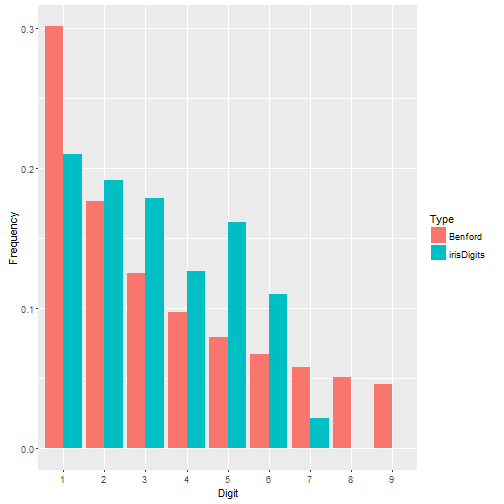# Some R Fundamentals

I recently came across the book R Programming for Bioinformatics at my local library and decided to check it out. I don’t do bioinformatics and the book is a little old (published in 2009), but I figured I would browse through it anyway. Chapter 2 is titled R Language Fundamentals. As I was flipping through it I found several little nuggets of information that I had either forgotten about over the years or never knew in the first place. I decided to document them here.

Variable names

Variable names cannot begin with a digit or underscore, and if they begin with a period they cannot be followed by a number. But we can bend these rules by quoting the names with backticks.

_evil <- "probably not wise"
_evil
##  "probably not wise"
.666_number of the beast <- sqrt(666^2)
.666_number of the beast
##  666
rm(_evil, .666_number of the beast)

Attributes

Attributes can be attached to any R object except NULL. They can be useful for storing metadata among many other things. For example, add a source for a dataset.

d <- VADeaths
attr(d, "source") <- "Molyneaux, L., Gilliam, S. K., and Florant, L. C.(1947) Differences in Virginia death rates by color, sex, age, and rural or urban residence. American Sociological Review, 12, 525–535."

To see the source:

attr(d, "source")
##  "Molyneaux, L., Gilliam, S. K., and Florant, L. C.(1947) Differences in Virginia death rates by color, sex, age, and rural or urban residence. American Sociological Review, 12, 525–535."

To see all attributes of an object:

attributes(d)
## $dim ##  5 4 ## ##$dimnames
## $dimnames[] ##  "50-54" "55-59" "60-64" "65-69" "70-74" ## ##$dimnames[]
##  "Rural Male"   "Rural Female" "Urban Male"   "Urban Female"
##
##
## $source ##  "Molyneaux, L., Gilliam, S. K., and Florant, L. C.(1947) Differences in Virginia death rates by color, sex, age, and rural or urban residence. American Sociological Review, 12, 525–535." To remove an attribute: attr(d, "source") <- NULL Not all attributes are displayed when called on an object. For example, after fitting a linear model, it appears there are only two attributes. m <- lm(dist ~ speed, data = cars) attributes(m) ##$names
##   "coefficients"  "residuals"     "effects"       "rank"
##   "fitted.values" "assign"        "qr"            "df.residual"
##   "xlevels"       "call"          "terms"         "model"
##
## $class ##  "lm" However, elements of the model object also have attributes. For example, the terms element has 10 attributes. out <- attributes(m$terms)
length(out)
##  10
names(out)
##   "variables"    "factors"      "term.labels"  "order"        "intercept"
##   "response"     "class"        ".Environment" "predvars"     "dataClasses"
attr(m$terms, "factors") ## speed ## dist 0 ## speed 1 The colon operator I often forget the colon operator can work with decimal values. 2.5:10.5 ##  2.5 3.5 4.5 5.5 6.5 7.5 8.5 9.5 10.5 And can go backwards: 10.2:1.2 ##  10.2 9.2 8.2 7.2 6.2 5.2 4.2 3.2 2.2 1.2 zero length vectors The sum of zero length vector is 0, but the product of a zero length vector is 1. x <- numeric() length(x) ##  0 sum(x) ##  0 prod(x) ##  1 This is ensures expected behavior when working with sums and products: # 12 + 0 sum(12, x) ##  12 # 12 * 1 prod(12, x) ##  12 .Machine The .Machine variable holds information about the numerical characteristics of your machine. For example, the largest integer my machine can represent: .Machine$integer.max
##  2147483647

If I add 1 to that, the result is numeric, not an integer.

x <- .Machine$integer.max x2 <- x + 1 is.integer(x2) ##  FALSE If I add 1L (an explicit integer) to that, the result is a warning and a NA. My machine cannot represent that integer. x2 <- x + 1L ## Warning in x + 1L: NAs produced by integer overflow x2 ##  NA Recoding factors There are several convenience functions in other packages for recoding variables such as recode in the {car} package, case_when in {dplyr}, and a bunch of functions in the {forcats} package. But it’s good to remember how to use base R to recode factors. Create a list with the recoding definitions and assign to the levels of the factor. g <- sample(letters[1:5], 30, replace = TRUE) g <- factor(g) g ##  e c d c d c b a e c a d e e d b e c b c b c b d d c b a d e ## Levels: a b c d e Put “a” and “b” into one group, “c” and “d” into another group, and keep “e” in it’s own group. lst <- list("A" = c("a", "b"), "B" = c("c", "d"), "C" = "e") levels(g) <- lst g ##  C B B B B B A A C B A B C C B A C B A B A B A B B B A A B C ## Levels: A B C If we like we can add an attribute to store the definition. attr(g, "recoding") <- c("A = {ab}, B = {cd}, C = {e}") g ##  C B B B B B A A C B A B C C B A C B A B A B A B B B A A B C ## attr(,"recoding") ##  A = {ab}, B = {cd}, C = {e} ## Levels: A B C lists can have dimensions Something more interesting than applicable is that lists can have dimensions. M <- as.table(rbind(c(762, 327, 468), c(484, 239, 477))) Xsq <- chisq.test(M) # produces 9 element list Xsq <- unclass(Xsq) # remove htest class dim(Xsq) <- c(3,3) Xsq ## [,1] [,2] [,3] ## [1,] 30.07015 "Pearson's Chi-squared test" numeric,6 ## [2,] 2 "M" table,6 ## [3,] 2.953589e-07 table,6 table,6 Xsq[1,3] ## [] ## A B C ## A 703.6714 319.6453 533.6834 ## B 542.3286 246.3547 411.3166 Environments We are not restricted to creating objects in the Global Environment. We can create our own environments using the new.env() function and then create objects in that environment. We can use the dollar sign operator or the assign() function. e1 <- new.env() e1$mod <- lm(dist ~ speed, data = cars)
e1$cumTotal <- function(x)tail(cumsum(x), n = 1) assign("vals", c(20, 23, 34, 19), envir = e1) ls(e1) ##  "cumTotal" "mod" "vals" ls() # list objects in Global Environment ##  "d" "e1" "g" "lst" "m" "M" "out" "x" "x2" "Xsq" We can access objects in our environment using the dollar sign operator or the get() and mget() functions. e1$cumTotal(c(2,4,6))
##  12
get("vals", envir = e1)
##  20 23 34 19
mget(c("mod", "vals"), envir = e1) # get more than one object
## $mod ## ## Call: ## lm(formula = dist ~ speed, data = cars) ## ## Coefficients: ## (Intercept) speed ## -17.579 3.932 ## ## ##$vals
##  20 23 34 19

We can save the environment and reload it in a future session.

save(e1, file = "e1.Rdata")
rm(e1)
load(file = "e1.Rdata")

We can also change the environment associated with an object that was created in the Global Environment.

f <- function(x)(vals + 1000) # vals object defined in e1 environment
environment(f) <- e1
f
## function(x)(vals + 1000)
## <environment: 0x000001b5ec98d4e0>
f()
##  1020 1023 1034 1019

Notice if we remove the environment using rm(), the function still remains in that environment and we have access to its objects

rm(e1)
f
## function(x)(vals + 1000)
## <environment: 0x000001b5ec98d4e0>
f()
##  1020 1023 1034 1019

rm(e1) simply removes the binding between the symbol “e1” and structure that contains the objects. Since the environment can be reached as the environment of f(), it remains available.

Brackets and Dollar Signs

I found this sentence enlightening: “One way of describing the behavior of the single bracket operator is that the type of the return value matches the type of the value it is applied to.” (p. 28) I like this in favor of metaphors involving trains.

lst <- list(a1 = 1:5, b = c("d", "g"), c = 99)
lst["a1"] # returns a list
## $a1 ##  1 2 3 4 5 [[ and $ extract single values.

lst[["a1"]]
##  1 2 3 4 5
lst$a1 ##  1 2 3 4 5 The $ operator supports partial matching.

lst$a ##  1 2 3 4 5 The [ and [[ operators support expressions, but not partial matching. ans <- "c" lst[ans] ##$c
##  99
lst[[ans]]
##  99

If names are duplicated in named vectors, then only the value corresponding to the first one is returned when subsetting with brackets.

x <- c("a" = 1, "a" = 2)
x["a"]
## a
## 1

The %in% operator can be useful to get all elements with the same name.

x[names(x) %in% "a"]
## a a
## 1 2

Matrix indexing

I don’t work with arrays that often, but when I do I often forget that I can index them with a matrix. Below I extract the value in row 1, column 4, from each of the 3 layers of the iris3 array.

m <- matrix(c(1,4,1,
1,4,2,
1,4,3),
ncol = 3, byrow = TRUE)
iris3[m]
##  0.2 1.4 2.5

Of course we can get the same result (in this case) using subsetting indices.

iris3[1,4,]
##     Setosa Versicolor  Virginica
##        0.2        1.4        2.5

Negative subscripts

Negative subscripts can appear on the left side of assignment.

x <- 1:10
x[-(2:4)] <- 99
x
##   99  2  3  4 99 99 99 99 99 99

Subsetting without dimensions

Use empty double brackets to select all elements and not change any attributes.

x <- matrix(10:1, ncol = 2)
x
##      [,1] [,2]
## [1,]   10    5
## [2,]    9    4
## [3,]    8    3
## [4,]    7    2
## [5,]    6    1
x[] <- sort(x)
x
##      [,1] [,2]
## [1,]    1    6
## [2,]    2    7
## [3,]    3    8
## [4,]    4    9
## [5,]    5   10

# rms for the rest of us

For a few years now I’ve been chipping away at Regression Modeling Strategies by Frank Harrell. Between the exposition, the case studies, the notes, the references, the code and exercises, it’s a deep and rich source of statistical learning. The book’s associated R package, {rms}, is used throughout the book and is one of the reasons it can be tough sledding at times, especially if you’re like me and learned regression using the base R functions lm() and glm(). It’s not that {rms} is hard to use. In fact it’s quite easy to use. But using functions such as summary() and anova() with {rms} models produces very different output than what you get with a base R lm() model and can seem baffling to the uninitiated. In this post I hope to explain a little of what {rms} is doing by comparing it to the more traditional approaches in R so commonly taught in classrooms and textbooks.

## Model fitting and summary output

To begin, let’s load the gala data from the {faraway} package. The gala data set contains data on species diversity on the Galapagos Islands. Below we use the base R lm() function to model the number of plant Species found on the island as function of the Area of the island (km$$^2$$), the highest Elevation of the island (m), the distance from the Nearest island (km), the distance from Santa Cruz island (km), and the area of the Adjacent island (square km). This is how I and thousands of others learned to do regression in R. Of course we use the familiar summary() function on our model object to see the model coefficients, marginal tests, the residual standard error, R squared, etc.

library(faraway)
data("gala")
m <- lm(Species ~ Area + Elevation + Nearest + Scruz + Adjacent,
data = gala)
summary(m)
##
## Call:
## lm(formula = Species ~ Area + Elevation + Nearest + Scruz + Adjacent,
##     data = gala)
##
## Residuals:
##      Min       1Q   Median       3Q      Max
## -111.679  -34.898   -7.862   33.460  182.584
##
## Coefficients:
##              Estimate Std. Error t value Pr(>|t|)
## (Intercept)  7.068221  19.154198   0.369 0.715351
## Area        -0.023938   0.022422  -1.068 0.296318
## Elevation    0.319465   0.053663   5.953 3.82e-06 ***
## Nearest      0.009144   1.054136   0.009 0.993151
## Scruz       -0.240524   0.215402  -1.117 0.275208
## Adjacent    -0.074805   0.017700  -4.226 0.000297 ***
## ---
## Signif. codes:  0 '***' 0.001 '**' 0.01 '*' 0.05 '.' 0.1 ' ' 1
##
## Residual standard error: 60.98 on 24 degrees of freedom
## Multiple R-squared:  0.7658, Adjusted R-squared:  0.7171
## F-statistic:  15.7 on 5 and 24 DF,  p-value: 6.838e-07

Now let’s fit the same model using the {rms} package. For this we use the ols() function. Notice we can use R’s formula syntax as usual. One additional argument we need to use that will come in handy in a few moments is x = TRUE. This stores the predictor variables with the model fit as a design matrix. Notice we don’t need to call summary() on the saved model object. We simply print it.

library(rms)
mr <- ols(Species ~ Area + Elevation + Nearest + Scruz + Adjacent,
data = gala, x = TRUE)
mr
## Linear Regression Model
##
## ols(formula = Species ~ Area + Elevation + Nearest + Scruz +
##     Adjacent, data = gala, x = TRUE)
##
##                  Model Likelihood    Discrimination
##                        Ratio Test           Indexes
## Obs       30    LR chi2     43.55    R2       0.766
## sigma60.9752    d.f.            5    R2 adj   0.717
## d.f.      24    Pr(> chi2) 0.0000    g      105.768
##
## Residuals
##
##      Min       1Q   Median       3Q      Max
## -111.679  -34.898   -7.862   33.460  182.584
##
##
##           Coef    S.E.    t     Pr(>|t|)
## Intercept  7.0682 19.1542  0.37 0.7154
## Area      -0.0239  0.0224 -1.07 0.2963
## Elevation  0.3195  0.0537  5.95 <0.0001
## Nearest    0.0091  1.0541  0.01 0.9932
## Scruz     -0.2405  0.2154 -1.12 0.2752
## Adjacent  -0.0748  0.0177 -4.23 0.0003

The coefficient tables and summaries of residuals are the same. Likewise both return R-squared and Adjusted R-squared. The residual standard error from the lm() output is called sigma in the ols() output.

The ols output also includes a “g” statistic. This is Gini’s mean difference and measures the dispersion of predicted values. It’s an alternative to standard deviation. We can use the {Hmisc} function GiniMd to calculate Gini’s mean difference for the model fit with lm() as follows.

Hmisc::GiniMd(predict(m))
##  105.7677

Whereas the summary output for lm() includes an F test for the null that all of the predictor coefficients equal 0, the ols() function reports a Likelihood Ratio Test. This tests the same null hypothesis using a ratio of likelihoods. We can calculate this test for the lm() model using the logLik() function. First we subtract the log likelihood of a model with no predictors from the log likelihood for the original model, and then multiply by 2. Apparently, according to Wikipedia, multiplying by 2 ensures mathematically that the test statistic converges to a chi-square distribution if the null is true. If the null is true, we expect this different of log likelihoods (or ratio, according to the quotient rule of logs) to be about 1. This statistic is very large. Clearly at least one of the predictor coefficients is not 0.

LRchi2 <- (logLik(m) - logLik(lm(gala$Species ~ 1)))*2 LRchi2 ## 'log Lik.' 43.55341 (df=7) If we wanted to calculate the p-value that appears in the ols() output, we can use the pchisq() function. Obviously the p-value in the ols() output is rounded. pchisq(LRchi2, df = 7, lower.tail = FALSE) ## 'log Lik.' 2.60758e-07 (df=7) ## Partial F tests and important measures Now let’s use the anova() function on both model objects and compare the output. For the lm() object, we get sequential partial F tests using Type I sums of squares. Each line tests the null hypothesis that adding the listed predictor to the previous model without it explains no additional variability. So the Area line compares a model with just an intercept to a model with an intercept and Area. The Elevation line compares a model with an intercept and Area to a model with an intercept, Area, and Elevation. And so on. Small p-values are evidence against the null. anova(m) ## Analysis of Variance Table ## ## Response: Species ## Df Sum Sq Mean Sq F value Pr(>F) ## Area 1 145470 145470 39.1262 1.826e-06 *** ## Elevation 1 65664 65664 17.6613 0.0003155 *** ## Nearest 1 29 29 0.0079 0.9300674 ## Scruz 1 14280 14280 3.8408 0.0617324 . ## Adjacent 1 66406 66406 17.8609 0.0002971 *** ## Residuals 24 89231 3718 ## --- ## Signif. codes: 0 '***' 0.001 '**' 0.01 '*' 0.05 '.' 0.1 ' ' 1 Calling anova() on the ols() object produces a series of partial F tests using Type II sums of squares. Each line tests the null hypothesis that adding the listed predictor to a model with all the other listed predictors already in it explains no additional variability. So the Area line compares a model with all the other predictors to a model with all the other predictors and Area. The Elevation line compares a model with all the other predictors to a model with all the other predictors and Elevation. The line labeled REGRESSION is the F Test reported in the summary output for the lm() model. anova(mr)  ## Analysis of Variance Response: Species ## ## Factor d.f. Partial SS MS F P ## Area 1 4.237718e+03 4.237718e+03 1.14 0.2963 ## Elevation 1 1.317666e+05 1.317666e+05 35.44 <.0001 ## Nearest 1 2.797576e-01 2.797576e-01 0.00 0.9932 ## Scruz 1 4.635787e+03 4.635787e+03 1.25 0.2752 ## Adjacent 1 6.640639e+04 6.640639e+04 17.86 0.0003 ## REGRESSION 5 2.918500e+05 5.837000e+04 15.70 <.0001 ## ERROR 24 8.923137e+04 3.717974e+03 To run these same Partial F tests for the lm() object we can use the base R drop1() function. drop1(m, test = "F") ## Single term deletions ## ## Model: ## Species ~ Area + Elevation + Nearest + Scruz + Adjacent ## Df Sum of Sq RSS AIC F value Pr(>F) ## <none> 89231 251.93 ## Area 1 4238 93469 251.33 1.1398 0.2963180 ## Elevation 1 131767 220998 277.14 35.4404 3.823e-06 *** ## Nearest 1 0 89232 249.93 0.0001 0.9931506 ## Scruz 1 4636 93867 251.45 1.2469 0.2752082 ## Adjacent 1 66406 155638 266.62 17.8609 0.0002971 *** ## --- ## Signif. codes: 0 '***' 0.001 '**' 0.01 '*' 0.05 '.' 0.1 ' ' 1 The {rms} package offers a convenient plot method for anova() objects that “draws dot charts depicting the importance of variables in the model” (from the anova.rms help page). The default importance measure is the chi-square statistic for each factor minus its degrees of freedom. There are several other importance measures available. See the anova.rms help page for the what argument. plot(anova(mr))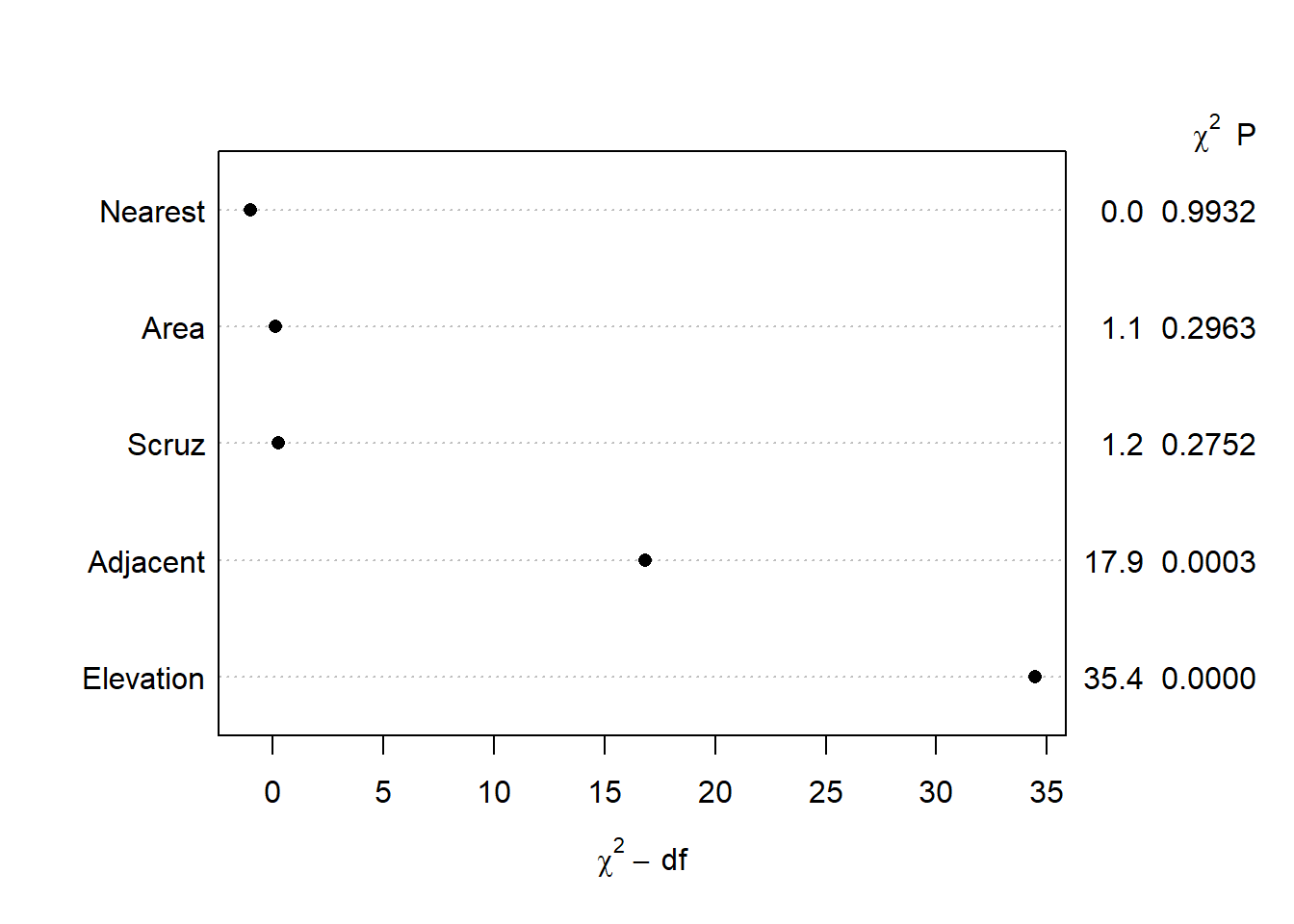## IQR effect plots Earlier we noted that we don’t use the summary() function on ols() model objects to see a model summary. That doesn’t mean there isn’t a summary() method available. There is, but it does something entirely different than the summary method for lm() objects. If we try it right now we get an error message: summary(mr) ## Error in summary.rms(mr): adjustment values not defined here or with datadist for Area Elevation Nearest Scruz Adjacent Notice what it says: “adjustment values not defined here or with datadist”. This tells us we need to define “adjustment values” to use the summary() function. Instead of summarizing the model, the {rms} summary function when called on {rms} model objects returns a “summary of the effects of each factor”. Let’s demonstrate what this means. The easiest way to define adjustment values is to use the datadist() function on our data frame and then set the global datadist option using the base R options() function. Harrell frequently assigns datadist results to “d” so we do the same. (Also, the help page for datadist states, “The best method is probably to run datadist once before any models are fitted, storing the distribution summaries for all potential variables.” I elected to wait for presentation purposes.) d <- datadist(gala) options(datadist = "d") If we print “d” we see adjustment levels for all variables in the model. d ## Species Endemics Area Elevation Nearest Scruz Adjacent ## Low:effect 13.0 7.25 0.2575 97.75 0.800 11.025 0.5200 ## Adjust to 42.0 18.00 2.5900 192.00 3.050 46.650 2.5900 ## High:effect 96.0 32.25 59.2375 435.25 10.025 81.075 59.2375 ## Low:prediction 2.0 1.45 0.0390 47.35 0.445 0.490 0.1000 ## High:prediction 319.1 85.40 782.6215 1229.40 40.205 193.905 782.6215 ## Low 2.0 0.00 0.0100 25.00 0.200 0.000 0.0300 ## High 444.0 95.00 4669.3200 1707.00 47.400 290.200 4669.3200 Now let’s call summary() on our {rms} model object and see what it returns. summary(mr) ## Effects Response : Species ## ## Factor Low High Diff. Effect S.E. Lower 0.95 Upper 0.95 ## Area 0.2575 59.238 58.980 -1.411900 1.3225 -4.1413 1.3176 ## Elevation 97.7500 435.250 337.500 107.820000 18.1110 70.4400 145.2000 ## Nearest 0.8000 10.025 9.225 0.084353 9.7244 -19.9860 20.1550 ## Scruz 11.0250 81.075 70.050 -16.849000 15.0890 -47.9910 14.2930 ## Adjacent 0.5200 59.238 58.718 -4.392400 1.0393 -6.5374 -2.2473 This produces an interquartile range “Effects” summary for all predictors. For example, in the first row we see the effect of Area is about -1.41. To calculate this we predict Species when Area = 59.238 (High, 75th percentile) and when Area = 0.2575 (Low, 25th percentile) and take the difference in predicted species. For each prediction, all other variables are held at their “Adjust to” level as shown above when we printed the datadist object. In addition to the effect, the standard error (SE) of the effect and a 95% confidence interval on the effect is returned. In this case we’re not sure if the effect is positive or negative. To calculate this effect measure using our lm() model object we can use the predict() function to make two predictions and then take the difference using the diff() function. Notice all the values in the newdata argument come from the datadist object above. The two values for Area are the “Low:effect” and “High:effect” values. The other values from the “Adjust to” row. # How to get Area effect in summary(mr) output = -1.411900 p <- predict(m, newdata = data.frame(Area = c(0.2575, 59.238), Elevation=97.75, Nearest=3.05, Scruz=46.65, Adjacent=2.59)) p ## 1 2 ## 26.90343 25.49153 diff(p) ## 2 ## -1.411895 To get the standard error and confidence interval we can do the following. (Recall 58.980 is the IQR of Area.) se <- sqrt((58.980 * vcov(m)["Area","Area"] * 58.980)) se ##  1.32247 diff(p) + c(-1,1) * se * qt(0.975, df = 24) ##  -4.141340 1.317549 This is made a little easier using the emmeans() function in the {emmeans} package. We use the at argument to specify at what values we wish to make predictions for Area. All other values are held at their median by setting cov.reduce = median. Sending the result to the {emmeans} function contrast with argument “revpairwise” says to subtract the estimated means in reverse order. Finally we pipe into the confint() to replicate the IQR effect estimate for Area that we saw in the ols() summary. library(emmeans) emmeans(m, specs = "Area", at = list(Area = c(0.2575, 59.238)), cov.reduce = median) |> contrast("revpairwise") |> confint() ## contrast estimate SE df lower.CL upper.CL ## Area59.238 - Area0.2575 -1.41 1.32 24 -4.14 1.32 ## ## Confidence level used: 0.95 There is also a plot method for {rms} summary objects. It plots the Effects and the 90, 95, and 99 percent confidence intervals using different shades of blue. Below we see the Area effect of -1.41 with relatively tight confidence intervals hovering around 0. plot(summary(mr))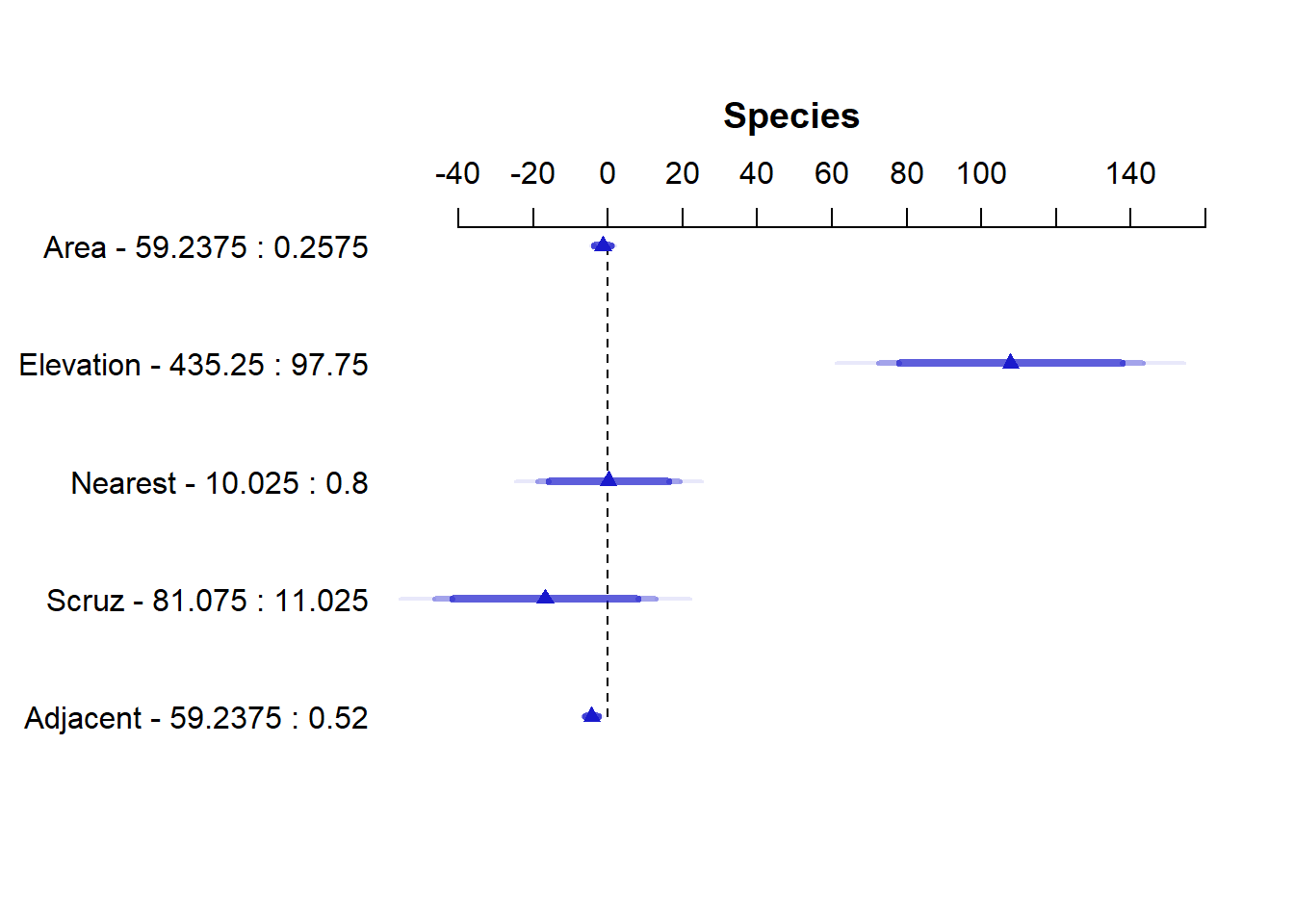## Non-linear effects Harrell advocates using non-linear effects in the form of regression splines. This makes a lot of sense when you pause and consider how many effects in real life are truly linear. Not many. Very few associations in nature indefinitely follow a straight line relationship. Fortunately, we can easily implement regression splines in R and specify how much non-linearity we want to entertain. We do this either in the form of degrees of freedom or knots. More of both means more non-linearity. I personally think of it as the number of times the relationship might change direction. One way to implement regression splines in R is via the ns() function in the {splines} package, which comes installed with R. Below we fit a model that allows the effect of Nearest to change directions three times by specifying df=3. We might think of this as sort of like using a 3-degree polynomial to model Nearest (but that’s not what we’re doing). The summary shows three coefficients for Nearest, neither of which have any interpretation. library(splines) m2 <- lm(Species ~ Area + Elevation + ns(Nearest, df = 3) + Scruz + Adjacent, data = gala) summary(m2) ## ## Call: ## lm(formula = Species ~ Area + Elevation + ns(Nearest, df = 3) + ## Scruz + Adjacent, data = gala) ## ## Residuals: ## Min 1Q Median 3Q Max ## -79.857 -28.285 -0.775 25.498 163.069 ## ## Coefficients: ## Estimate Std. Error t value Pr(>|t|) ## (Intercept) 13.18825 27.39386 0.481 0.6350 ## Area -0.03871 0.02098 -1.845 0.0785 . ## Elevation 0.35011 0.05086 6.883 6.51e-07 *** ## ns(Nearest, df = 3)1 -168.44016 68.41261 -2.462 0.0221 * ## ns(Nearest, df = 3)2 -56.97623 57.67426 -0.988 0.3339 ## ns(Nearest, df = 3)3 38.61120 46.22137 0.835 0.4125 ## Scruz -0.13735 0.19900 -0.690 0.4973 ## Adjacent -0.08254 0.01743 -4.734 0.0001 *** ## --- ## Signif. codes: 0 '***' 0.001 '**' 0.01 '*' 0.05 '.' 0.1 ' ' 1 ## ## Residual standard error: 55.11 on 22 degrees of freedom ## Multiple R-squared: 0.8247, Adjusted R-squared: 0.7689 ## F-statistic: 14.78 on 7 and 22 DF, p-value: 5.403e-07 To decide whether we should keep this non-linear effect, we could use the anova() function to compare this updated more complex model to the original model with only linear effects. The null of the test is that both models are equally adequate. It appears there is some evidence that this non-linearity improves the model. anova(m, m2) ## Analysis of Variance Table ## ## Model 1: Species ~ Area + Elevation + Nearest + Scruz + Adjacent ## Model 2: Species ~ Area + Elevation + ns(Nearest, df = 3) + Scruz + Adjacent ## Res.Df RSS Df Sum of Sq F Pr(>F) ## 1 24 89231 ## 2 22 66808 2 22424 3.6921 0.04144 * ## --- ## Signif. codes: 0 '***' 0.001 '**' 0.01 '*' 0.05 '.' 0.1 ' ' 1 To entertain non-linear effects in {rms} use the rcs() function, which stands for restricted cubic splines. Instead of degrees of freedom we specify knots. Three degrees of freedom corresponds to four knots. mr2 <- ols(Species ~ Area + Elevation + rcs(Nearest, 4) + Scruz + Adjacent, data = gala, x = TRUE) mr2 ## Linear Regression Model ## ## ols(formula = Species ~ Area + Elevation + rcs(Nearest, 4) + ## Scruz + Adjacent, data = gala, x = TRUE) ## ## Model Likelihood Discrimination ## Ratio Test Indexes ## Obs 30 LR chi2 51.32 R2 0.819 ## sigma55.9556 d.f. 7 R2 adj 0.762 ## d.f. 22 Pr(> chi2) 0.0000 g 107.069 ## ## Residuals ## ## Min 1Q Median 3Q Max ## -90.884 -29.870 -3.715 28.019 163.497 ## ## ## Coef S.E. t Pr(>|t|) ## Intercept 14.1147 30.2230 0.47 0.6451 ## Area -0.0368 0.0212 -1.74 0.0964 ## Elevation 0.3444 0.0512 6.73 <0.0001 ## Nearest 3.6081 16.9795 0.21 0.8337 ## Nearest' -714.5143 891.8922 -0.80 0.4316 ## Nearest'' 1001.4929 1207.4895 0.83 0.4158 ## Scruz -0.2286 0.1978 -1.16 0.2602 ## Adjacent -0.0806 0.0175 -4.60 0.0001 Notice we get three coefficients for Nearest that differ in value from our lm() result. A brief explanation of why that is can be found on datamethods.org. This paper provides a deeper explanation. Calling the {rms} anova() method on the model object returns a test for nonlinearity under the Nearest test, labeled “Nonlinear”. Notice the resulting p-value is slightly higher and exceeds 0.05. anova(mr2) ## Analysis of Variance Response: Species ## ## Factor d.f. Partial SS MS F P ## Area 1 9446.295 9446.295 3.02 0.0964 ## Elevation 1 141872.398 141872.398 45.31 <.0001 ## Nearest 3 20348.931 6782.977 2.17 0.1208 ## Nonlinear 2 20348.651 10174.326 3.25 0.0580 ## Scruz 1 4182.177 4182.177 1.34 0.2602 ## Adjacent 1 66204.433 66204.433 21.14 0.0001 ## REGRESSION 7 312198.651 44599.807 14.24 <.0001 ## ERROR 22 68882.715 3131.033 To replicate the {rms} anova() output using lm(), we need to change ns() arguments. The following R code comes from this hbiostat.org page w <- rcs(gala$Nearest, 4)
kn <- attr(w, 'parms')
m2 <- lm(Species ~ Area + Elevation + ns(Nearest, knots = kn[2:3],
Boundary.knots = c(kn, kn))
+ Scruz + Adjacent, data = gala)
anova(m, m2)
## Analysis of Variance Table
##
## Model 1: Species ~ Area + Elevation + Nearest + Scruz + Adjacent
## Model 2: Species ~ Area + Elevation + ns(Nearest, knots = kn[2:3], Boundary.knots = c(kn,
##     kn)) + Scruz + Adjacent
##   Res.Df   RSS Df Sum of Sq      F  Pr(>F)
## 1     24 89231
## 2     22 68883  2     20349 3.2495 0.05801 .
## ---
## Signif. codes:  0 '***' 0.001 '**' 0.01 '*' 0.05 '.' 0.1 ' ' 1

Which approach is better: ns() or rcs()? I don’t pretend to know and I’m not sure it really matters. The substance of the results doesn’t differ much. Yes, we saw a p-value jump from 0.04 to 0.05, but we know better than to make hard binary decisions based on a p-value.

## Effect plots

Since interpreting non-linear effect coefficients is all but impossible, we turn to visualization. When fitting a non-linear model with lm(), the {effects} and {ggeffects} packages are fantastic for creating effect plots.

m2 <- lm(Species ~ Area + Elevation + ns(Nearest, df = 3) +
library(effects)
Effect("Nearest", m2) |> plot()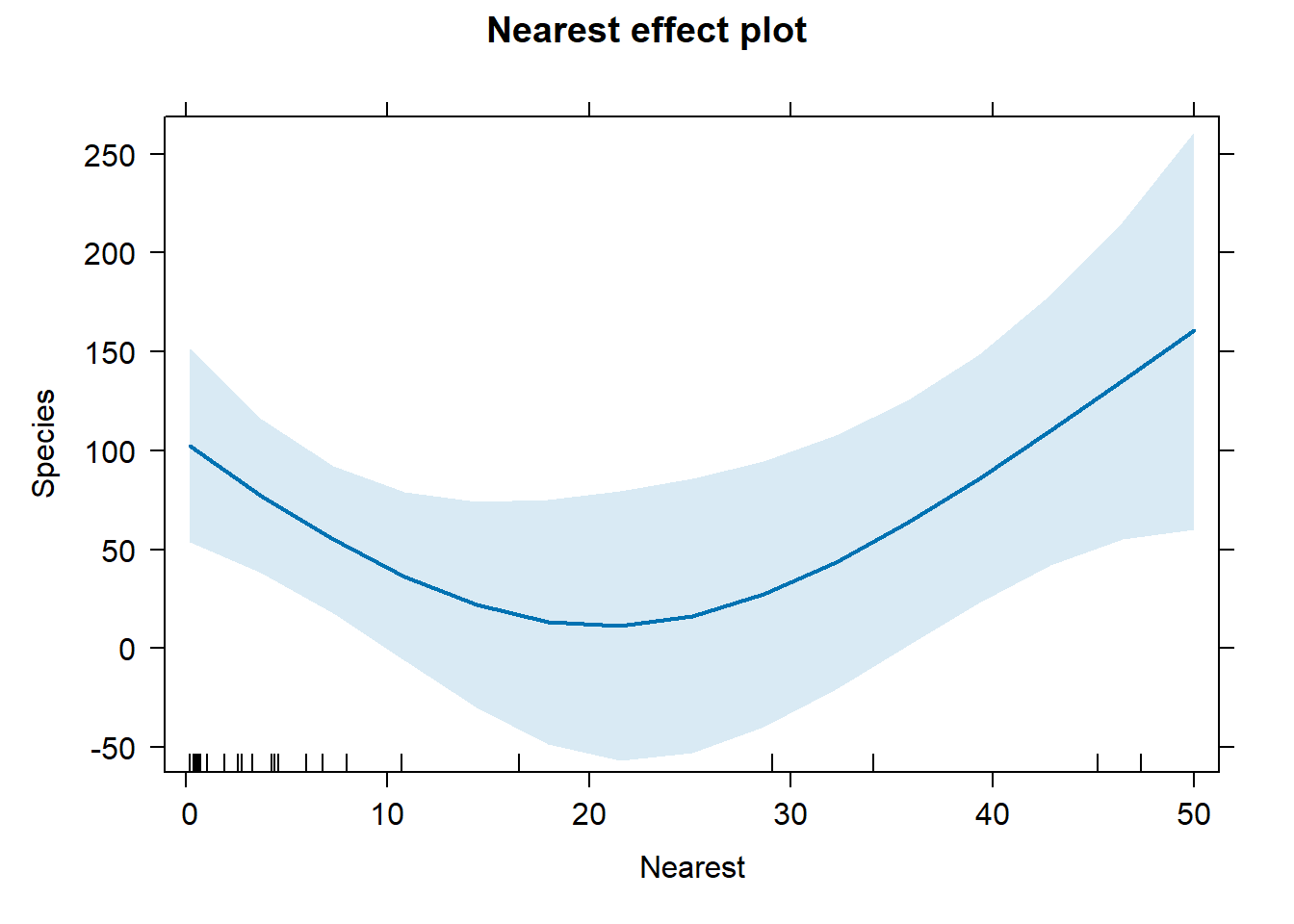library(ggeffects)
ggeffect(m2, "Nearest") |> plot()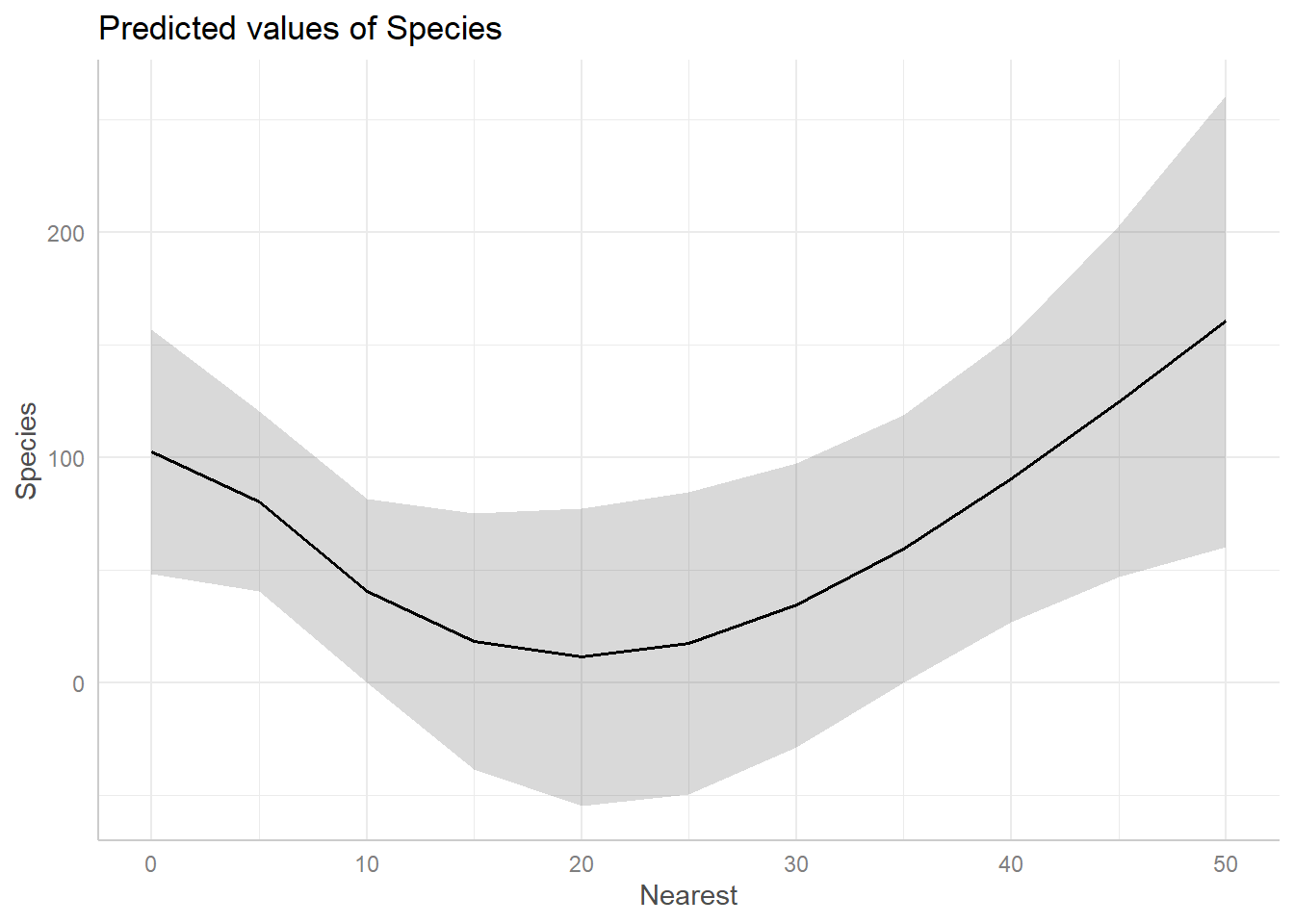In both cases we see the effect of Nearest is negative for values 0 – 20, but then seems to increase for values above 20.

To get a similar plot for an {rms} model, we can use the Predict() function (note the capital “P”; this is an {rms} function) and then pipe into plot(). You can also pipe into ggplot() and plotp() to get ggplot2 and plotly plots, respectively.

Predict(mr2, Nearest) |> plot()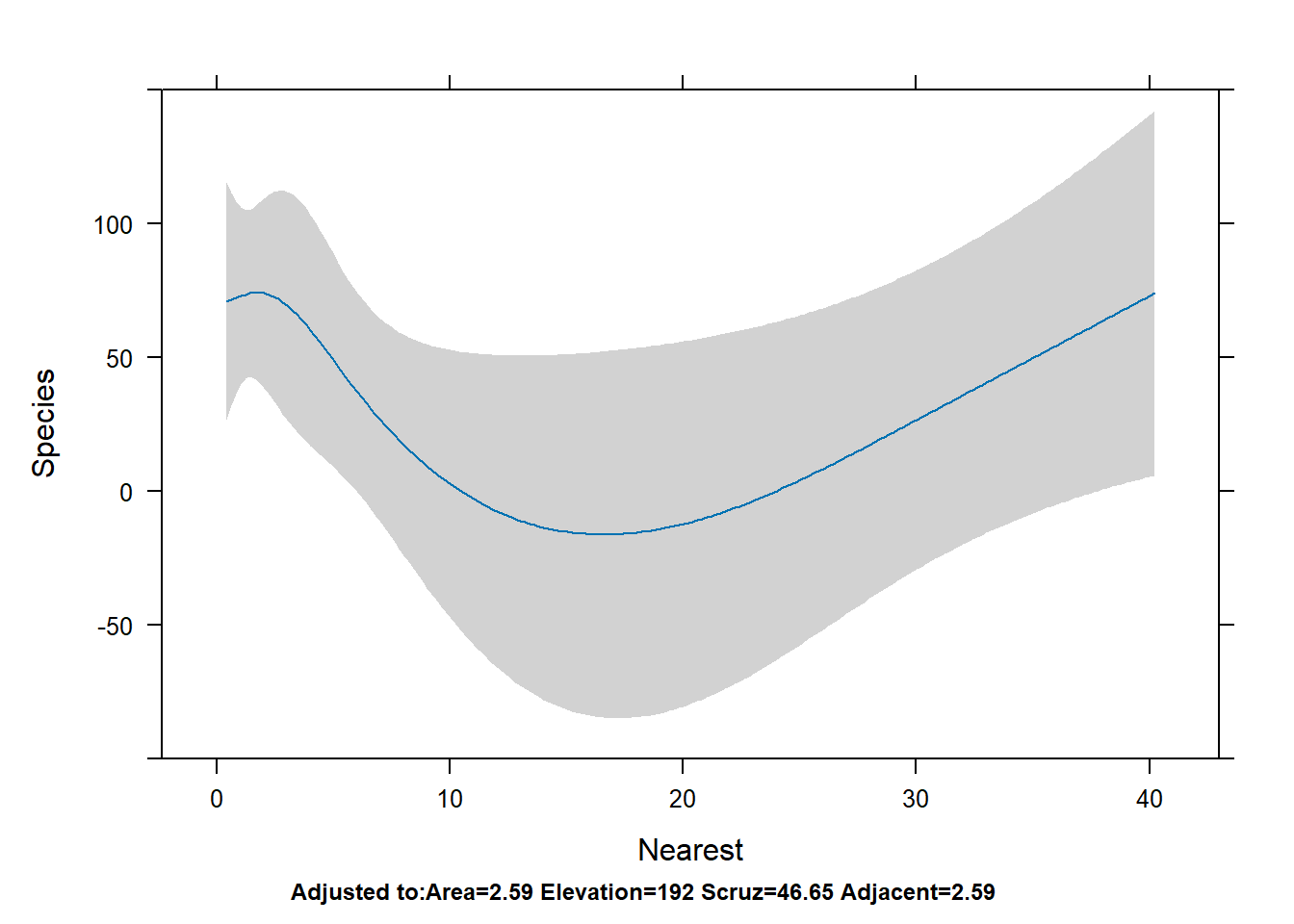To get all effect plots in one go, we can use the {effects} function allEffects() with the lm() model.

allEffects(m2) |> plot()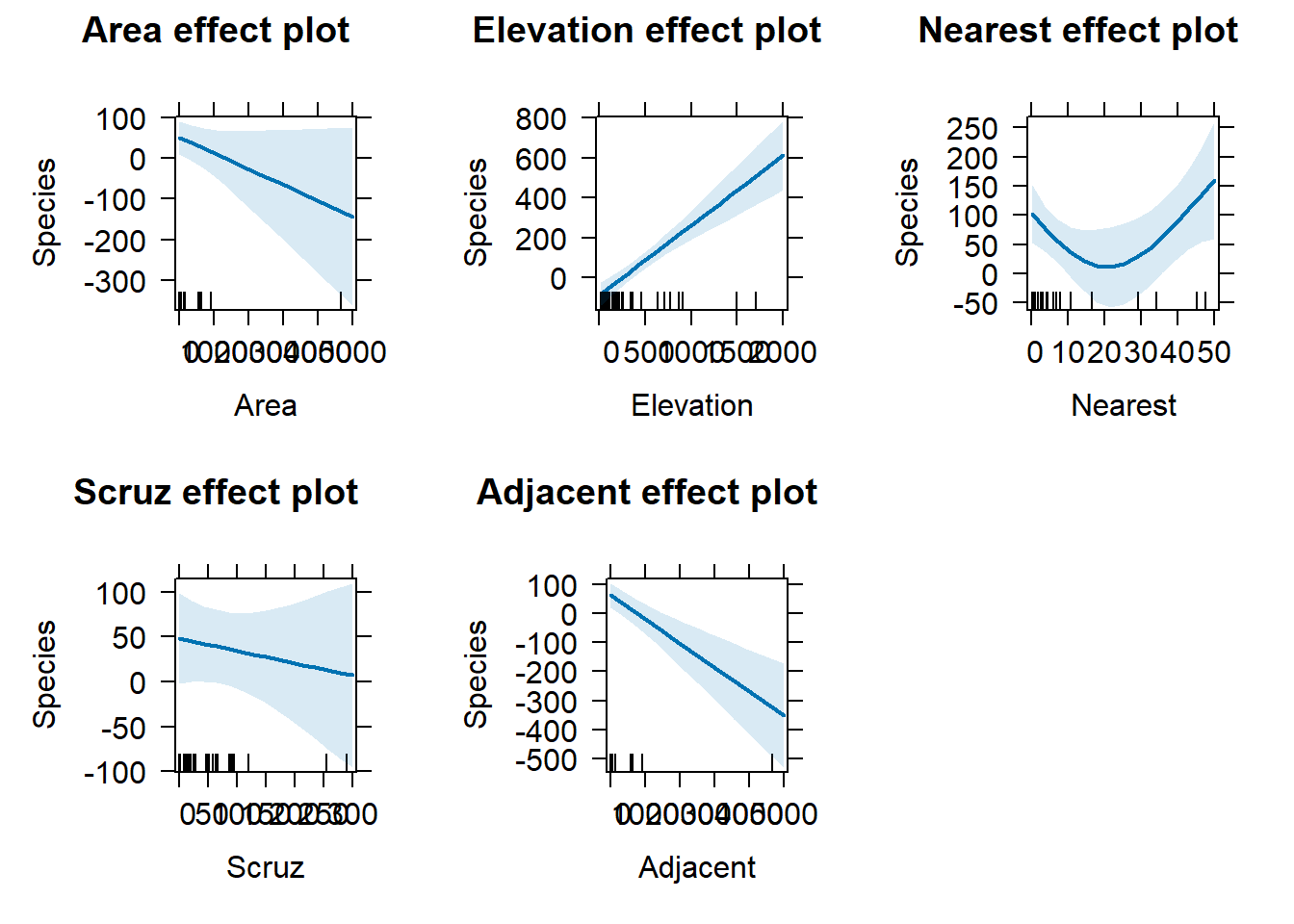To do the same with {rms}, we use the Predict() function with no predictors specified. Notice the y-axis has the same limits for all plots.

Predict(mr2) |> plot()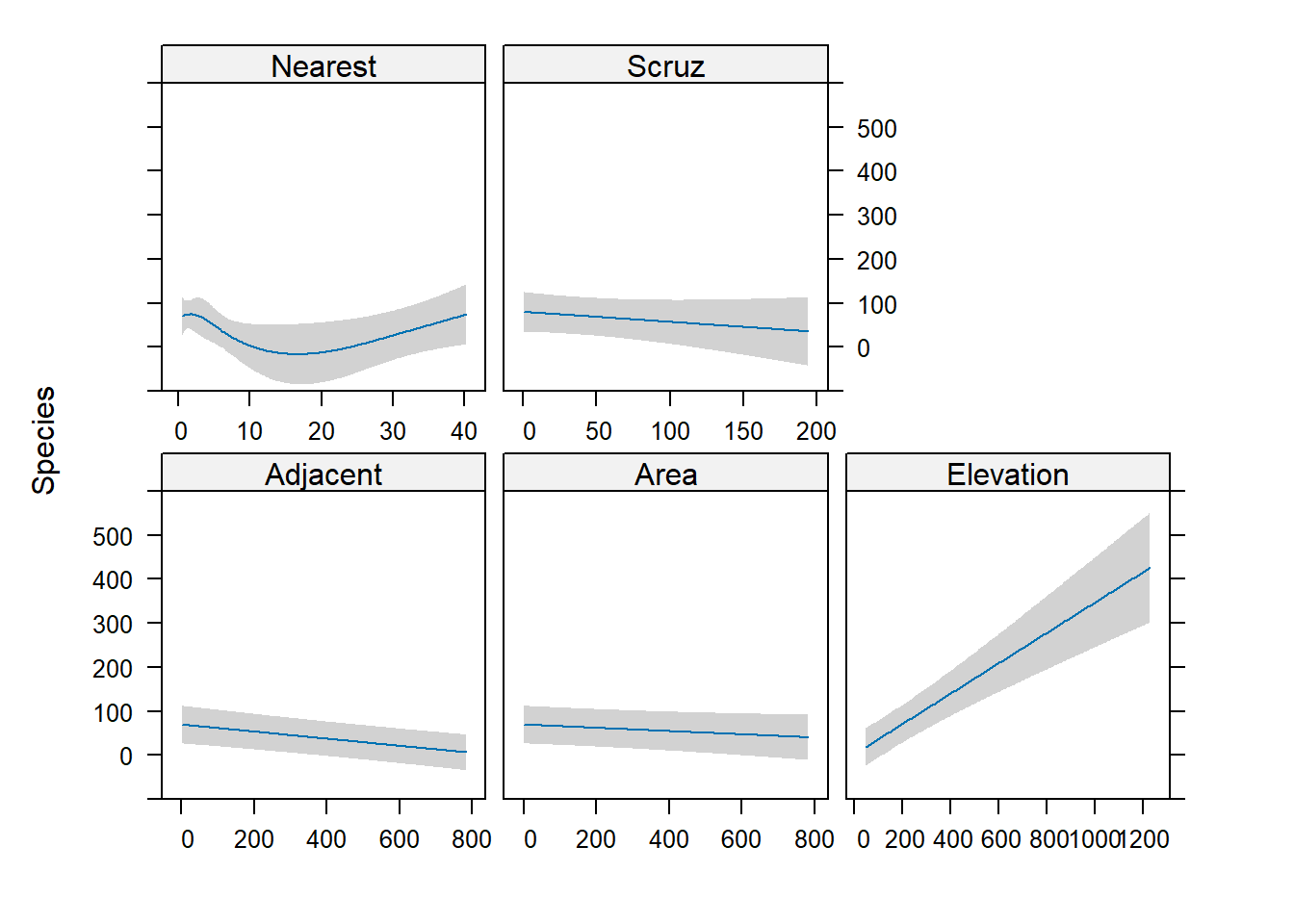## Diagnostics

A linear model makes several assumptions, two of which are constant variance of residuals and normality of residuals. The plot() method for lm() objects makes this easy to assess graphically.

op <- par(mfrow = c(2,2))
plot(m2)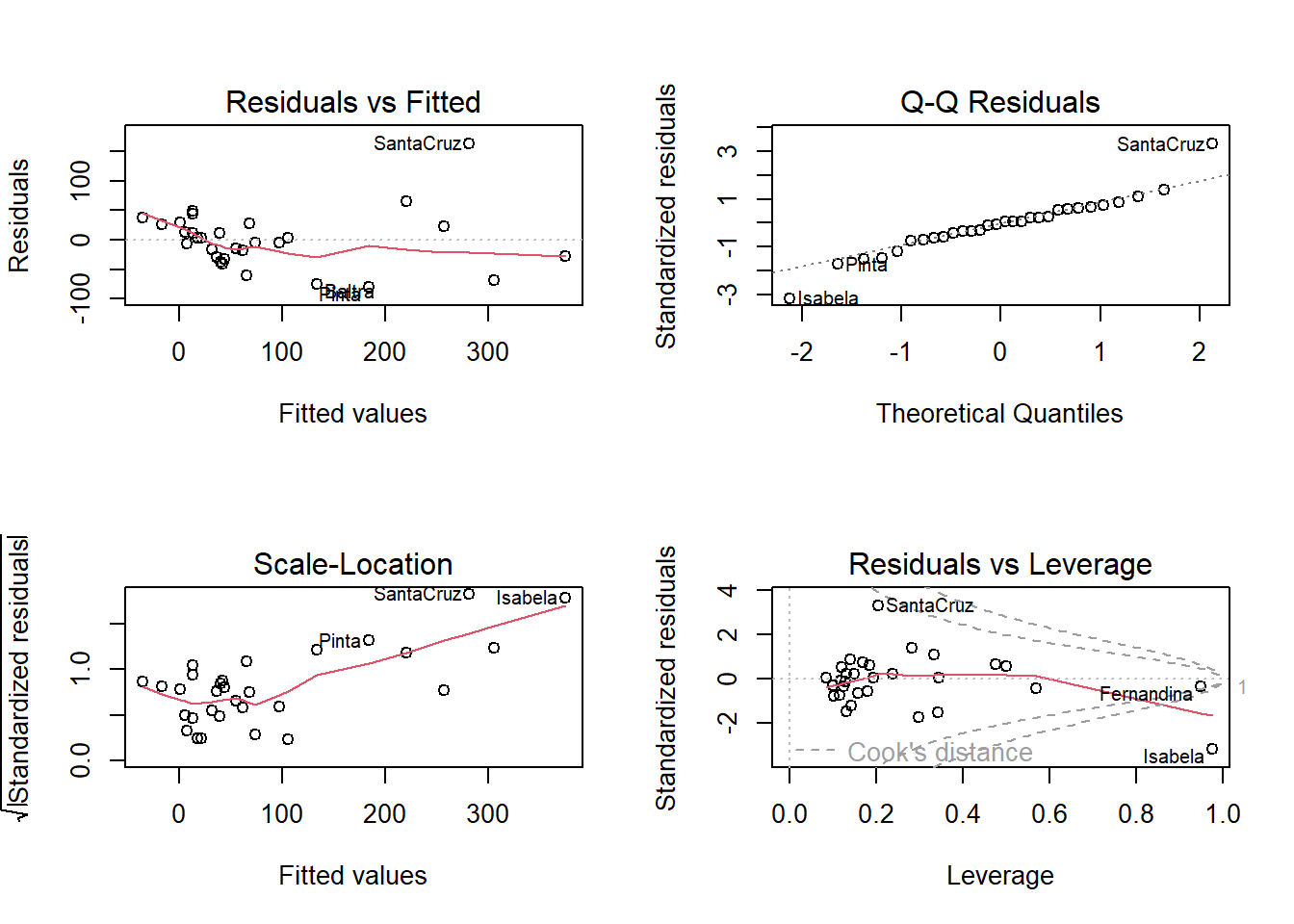par(op)

The left two plots assess constant variance using two different types of residuals. The upper right plot assess normality. The bottom right helps identify influential observations. The islands of Isabela and Santa Cruz stand out as either not being well fit by the model or unduly influencing the fit of the model.

We can use the plot.lm() method on the ols() model, but only the first one (i.e., which = 1).

plot(mr2, which = 1)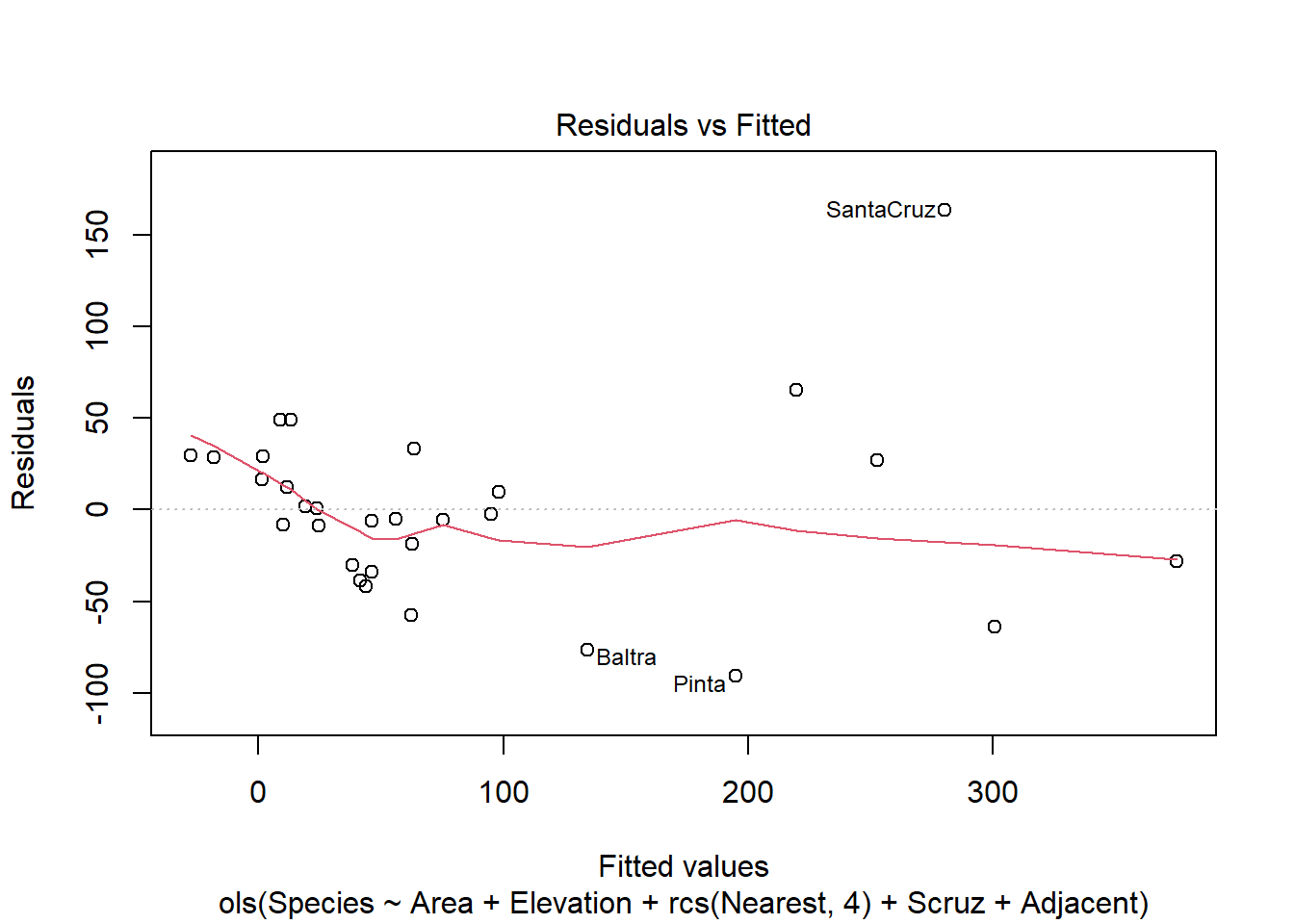To create a QQ plot to assess normality of residuals we need to extract the residuals using the residuals() function and plot with qqnorm() and qqline()

r <- residuals(mr2, type="student")
qqnorm(r)
qqline(r)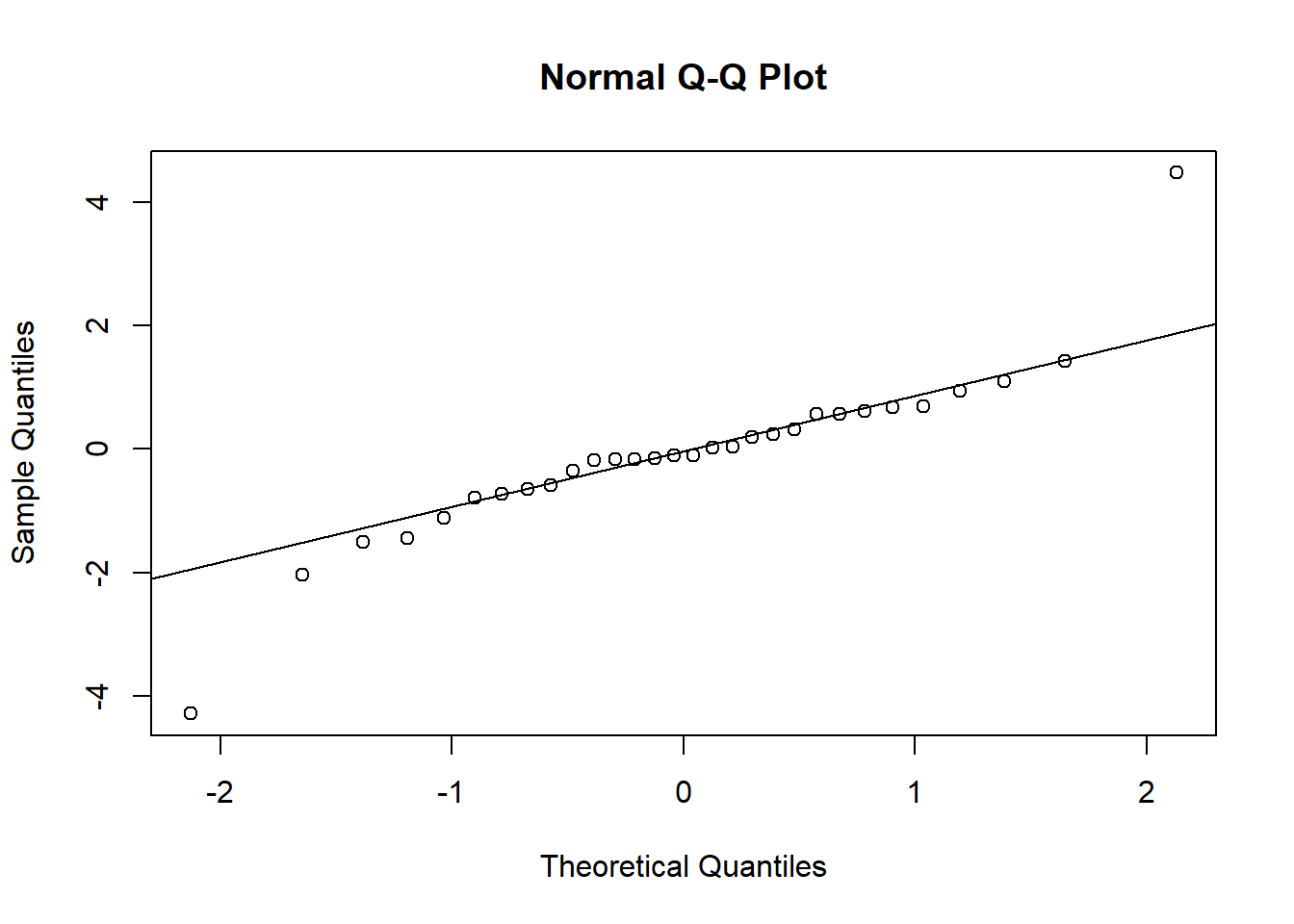To check for possible influential observations for an lm() model we can use the base R function influence.measures() and its associated summary() method. See ?influence.measures for a breakdown of what is returned. The columns that begin “dfb” are the DFBETA statistics. DFBETAS indicate the effect that deleting each observation has on the estimates of the regression coefficients. That’s why there’s a DFBETA for every coefficient in the model.

summary(influence.measures(m2))
## Potentially influential observations of
##   lm(formula = Species ~ Area + Elevation + ns(Nearest, df = 3) +      Scruz + Adjacent, data = gala) :
##
##              dfb.1_ dfb.Area dfb.Elvt dfb.n(N,d=3)1 dfb.n(N,d=3)2 dfb.n(N,d=3)3
## Darwin        -0.02   0.07    -0.08     0.05         -0.10         -0.10
## Fernandina     0.14   0.19    -0.15     0.02          0.00          0.03
## Genovesa      -0.07  -0.08     0.12     0.13         -0.15         -0.43
## Isabela        0.05 -18.76_*   4.55_*  -1.69_*       -1.12_*        0.94
## SanCristobal  -0.09  -0.11     0.18    -0.18          0.22          0.49
## SantaCruz      0.43  -1.03_*   1.58_*  -0.57         -0.96         -0.38
## Wolf           0.00   0.00     0.00     0.01          0.00          0.00
##              dfb.Scrz dfb.Adjc dffit    cov.r    cook.d   hat
## Darwin         0.48     0.00     0.62     2.36_*   0.05     0.48
## Fernandina    -0.07    -0.91    -1.51    27.71_*   0.30     0.95_*
## Genovesa       0.14    -0.10    -0.47     3.16_*   0.03     0.57
## Isabela       -0.54    -0.78   -26.78_*   0.45    50.94_*   0.98_*
## SanCristobal  -0.18    -0.12     0.58     2.56_*   0.04     0.50
## SantaCruz     -0.17    -1.16_*   2.33_*   0.01     0.36     0.21
## Wolf           0.03     0.00     0.04     2.21_*   0.00     0.34

The {rms} package offers the which.influence() and show.influence() tandem to identify observations (via DFBETAS) that effect regression coefficients with their removal. Below we set cutoff = 0.4. An asterisk is placed next to a variable when any of the coefficients associated with that variable change by more than 0.4 standard errors upon removal of the observation. Below we see that removing the Isabela observation changes four of the coefficients. (Also, this is why we set x = TRUE in our call to ols(), so we could use these functions.) The values displayed are the observed values for each observation.

w <- which.influence(mr2, cutoff = 0.4)
show.influence(w, gala)
##             Count     Area Elevation Nearest  Scruz Adjacent
## Darwin          1     2.33       168    34.1 *290.2     2.85
## Fernandina      1   634.49      1494     4.3   95.3 *4669.32
## Isabela         4 *4669.32     *1707     0.7 * 28.1 * 634.49
## Pinta           3 *  59.56     * 777    29.1  119.6 * 129.49
## SanSalvador     3 * 572.33     * 906     0.2   19.8 *   4.89
## SantaCruz       3 * 903.82     * 864     0.6    0.0 *   0.52
## SantaFe         1    24.08       259   *16.5   16.5     0.52
## SantaMaria      5 * 170.92     * 640   * 2.6   49.2 *   0.10

Got a question? Harrell runs a discussion board for the {rms} package.

Chapter 14 of these course notes by Thomas Love are also helpful.

And here’s a nice blog post called An Introduction to the Harrell“verse”: Predictive Modeling using the Hmisc and rms Packages by Nicholas Ollberding.

Eventually you may want to buy his book, Regression Modeling Strategies. It’s not cheap, but it’s not outrageous either. Considering how much information, advice, code, examples, and references it contains, I think it’s a bargain. It’s a text that will provide many months, if not years, of study.

# Would you rather

Someone texted me the following image and asked for my response.So what would I rather do?

• Flip 3 coins and win if all match
• Roll 3 dice and win if none match

I would rather do the one with the highest probability of happening. So let’s calculate that using R.

There are two ways to solve problems like this. One is to use the appropriate probability distribution and calculate it mathematically. The other is to enumerate all possible outcomes and find the proportion of interest. Let’s do the latter first.

We’ll use the expand.grid function to create a data frame of all possible outcomes of flipping 3 coins. All we have to do is give it 3 vectors representing coins. It’s such a small sample space we can eyeball it and see the probability of getting all heads or all tails is 2/8 or 0.25.

s1 <- expand.grid(c("H", "T"), c("H", "T"), c("H", "T"))
s1
##   Var1 Var2 Var3
## 1    H    H    H
## 2    T    H    H
## 3    H    T    H
## 4    T    T    H
## 5    H    H    T
## 6    T    H    T
## 7    H    T    T
## 8    T    T    T

If we wanted to use R to determine the proportion, we could use apply to apply a function to each row and return the number of unique values, and then find the proportion of 1s. (1 means there was only one unique value: all “H” or all “T”)

count1 <- apply(s1, 1, function(x)length(unique(x)))
mean(count1 == 1)
##  0.25

We can do the same with rolling 3 dice. Give expand.grid 3 dice and have it generate all possible results. This will generate 216 possibilities, so there’s no eyeballing this for an answer. As before, we’ll apply a function to each row and determine if there are any duplicates. The anyDuplicated function returns the location of the first duplicate within a vector. If there are no duplicates, the result is a 0, which means we just need to find the proportion of 0s.

s2 <- expand.grid(1:6, 1:6, 1:6)
count2 <- apply(s2, 1, anyDuplicated)
mean(count2 == 0)
##  0.5555556

An easier way to do that is to use permutation calculations. There are $$6^3 = 216$$ possible results from rolling 3 dice. Furthermore there are $$6 \cdot 5 \cdot 4 = 120$$ possible non-matching results. There are 6 possibilities for the first die, only 5 for the second, and 4 for the third. We can then divide to find the probability: $$120/216 = 0.55$$.

Clearly we’d rather roll the dice (assuming fair coins and fair dice).

We can also answer the question using the binomial probability distribution. The binomial distribution is appropriate when you have:

• two outcomes (Heads vs Tales, no match vs One or more match)
• independent events
• same probability for each event

The dbinom function calculates probabilities of binomial outcomes. Below we use it to calculate the probability of 0 heads (3 tails) and 3 heads, and sum the total. The x argument is the sum of “successes”, for example 0 heads (or tails, whatever you call a “success”). The size argument is the number of trials, or coins in this case. The prob argument is the probability of success on each trial, or for each coin in this case.

dbinom(x = 0, size = 3, prob = 0.5) +
dbinom(x = 3, size = 3, prob = 0.5)
##  0.25

We can also frame the dice rolling as a binomial outcome, but it’s a little trickier. Think of rolling the dice one at a time:

• It doesn’t matter what we roll the first time. We don’t care if it’s a 1 or 6 or whatever. We’re certain to get something, so the probability is 1.
• The second role is a “success” if it does not match the first role. That’s one dice roll (size = 1) where success (x = 1) happens with probability of 5/6.
• The third and final roll is a “success” if it doesn’t match either of the first two rolls. That’s one dice roll (size = 1) where success (x = 1) happens with probability of 4/6.

Notice we don’t add these probabilities but rather multiply them.

1 * dbinom(x = 1, size = 1, prob = 5/6) *
dbinom(x = 1, size = 1, prob = 4/6)
##  0.5555556

We added the coin flipping probabilities because they each represented mutually exclusive events. They cannot both occur. There is one way to get 0 successes and one way to get 3 successes. Together they sum to the probability of all matching (ie, all heads or all tales).

We multiplied the dice rolling probabilities because they each represented events that could occur at the same time, and they were conditional if we considered each die in turn. This requires the multiplication rule of probability.

# Fixing the p-value note in a HTML stargazer table

If you insert a stargazer table into R Markdown that outputs an HTML file, you may get a p-value note that looks like this:

p<0.1; p<0.05; p<0.01

What should be displayed is this:

*p<0.1; **p<0.05; ***p<0.01

This is the legend for understanding the “stars” in the regression table. What’s happening is the asterisks are being treated as markdown code, which is adding italics and bolding to the note instead of simply showing the asterisks verbatim. (Recall that in Markdown surrounding text with one asterisk adds italics and surrounding text with two asterisks adds bolding.)

To fix this we can add the following two arguments to the stargazer function:

notes.append = FALSE

notes = c("<sup>&sstarf;</sup>p<0.1; <sup>&sstarf;&sstarf;</sup>p<0.05; <sup>&sstarf;&sstarf;&sstarf;</sup>p<0.01")

&sstarf; is an HTML entity for a star character. notes.append = FALSE says to NOT append the note but rather replace the existing note. The notes argument specifies the note we want to add using the &sstarf; HTML entity.

# Most useful tests for an ANCOVA model

In his book Regression Modeling Strategies, 2nd Ed, Frank Harrell provides a list of what he calls the “most useful tests” for a 2-level factor $$\times$$ numeric model (Table 2.2, p. 19). This is often called an Analysis of Covariance, or ANCOVA. The basic idea is we have a numeric response with substantial variability and we seek to understand the variability by modeling the mean of the response as a function of a categorical variable and a numeric variable.

Let’s simulate some data for such a model and then see how we can use R to carry out these tests.

n <- 400
set.seed(1)
sex <- factor(sample(x = c("f", "m"), size = n, replace = TRUE))
age <- round(runif(n = n, min = 18, max = 65))
y <- 1 + 0.8*age + 0.4*(sex == "m") - 0.7*age*(sex == "m") + rnorm(n, mean = 0, sd = 8)
dat <- data.frame(y, age, sex)


The data contain a numeric response, y, that is a function of age and sex. I set the “true” coefficient values to 1, 0.8, 0.4, and -0.7. They correspond to $$\beta_0$$ through $$\beta_3$$ in the following model:

$y = \beta_0 + \beta_1 age + \beta_2 sex + \beta_3 age \times sex$

In addition the error component is a Normal distribution with a standard deviation of 8.

Now let’s model the data and see how close we get to recovering the true parameter values.

mod <- lm(y ~ age * sex, dat)
summary(mod)

##
## Call:
## lm(formula = y ~ age * sex, data = dat)
##
## Residuals:
##      Min       1Q   Median       3Q      Max
## -23.8986  -5.8552  -0.2503   6.0507  30.6188
##
## Coefficients:
##             Estimate Std. Error t value Pr(>|t|)
## (Intercept)  0.27268    1.93776   0.141    0.888
## age          0.79781    0.04316  18.484   <2e-16 ***
## sexm         2.07143    2.84931   0.727    0.468
## age:sexm    -0.72702    0.06462 -11.251   <2e-16 ***
## ---
## Signif. codes:  0 '***' 0.001 '**' 0.01 '*' 0.05 '.' 0.1 ' ' 1
##
## Residual standard error: 8.661 on 396 degrees of freedom
## Multiple R-squared:  0.7874, Adjusted R-squared:  0.7858
## F-statistic:   489 on 3 and 396 DF,  p-value: < 2.2e-16


While the coefficient estimates for age and the age $$\times$$ sex interaction are pretty close to the true values, the same cannot be said for the intercept and sex coefficients. The residual standard error of 8.661 is close to the true value of 8.

We can see in the summary output of the model that four hypothesis tests, one for each coefficient, are carried out for us. Each are testing if the coefficient is equal to 0. Of those four, only one qualifies as one of the most useful tests: the last one for age:sexm. This tests if the effect of age is independent of sex and vice versa. Stated two other ways, it tests if age and sex are additive, or if the age effect is the same for both sexes. To get a better understanding of what we’re testing, let’s plot the data with fitted age slopes for each sex.

library(ggplot2)
ggplot(dat, aes(x = age, y = y, color = sex)) +
geom_point() +
geom_smooth(method="lm")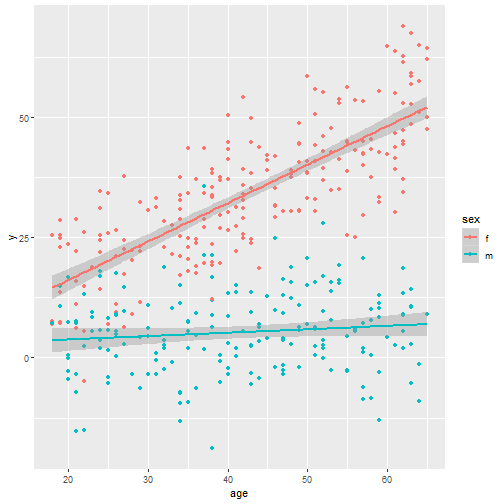Visually it appears the effect of age is not independent of sex. It seems more pronounced for females. Is this effect real or maybe due to chance? The hypothesis test in the summary output for age:sexm evaluates this. Obviously the effect seems very real. We are not likely to see such a difference in slopes this large if there truly was no difference. It does appear the effect of age is different for each sex. The estimate of -0.72 estimates the difference in slopes (or age effect) for the males and females.

The other three hypothesis tests are not very useful.

• Testing if the Intercept is 0 is testing whether y is 0 for females at age 0.
• Testing if age is 0 is testing whether age is associated with y for males.
• Testing if sexm is 0 is testing whether sex is associated with y for subjects at age 0.

Other more useful tests, as Harrell outlines in Table 2.2, are as follows:

• Is age associated with y?
• Is sex associated with y?
• Are either age or sex associated with y?

The last one is answered in the model output. That’s the F-statistic in the last line. It tests whether all coefficients (except the intercept) are equal to 0. The result of this test is conclusive. At least one of the coeffcients is not 0.

To test if age is associated with y, we need to test if both the age and age:sexm coefficents are equal to 0. The car package by John Fox provides a nice function for this purpose called linearHypothesis. It takes at least two arguments. The first is the fitted model object and the second is a vector of hypothesis tests. Below we specify we want to test if “age = 0” and “age:sexm = 0”

library(car)
linearHypothesis(mod, c("age = 0", "age:sexm = 0"))

## Linear hypothesis test
##
## Hypothesis:
## age = 0
## age:sexm = 0
##
## Model 1: restricted model
## Model 2: y ~ age * sex
##
##   Res.Df   RSS Df Sum of Sq      F    Pr(>F)
## 1    398 55494
## 2    396 29704  2     25790 171.91 < 2.2e-16 ***
## ---
## Signif. codes:  0 '***' 0.001 '**' 0.01 '*' 0.05 '.' 0.1 ' ' 1


The result is once again conclusive. The p-value is virtually 0. It does indeed appear that age is associated with y.

Likewise, to test if sex is associated with y, we need to test if both the sex and age:sexm coefficents are equal to 0.

linearHypothesis(mod, c("sexm = 0", "age:sexm = 0"))

## Linear hypothesis test
##
## Hypothesis:
## sexm = 0
## age:sexm = 0
##
## Model 1: restricted model
## Model 2: y ~ age * sex
##
##   Res.Df    RSS Df Sum of Sq     F    Pr(>F)
## 1    398 119354
## 2    396  29704  2     89651 597.6 < 2.2e-16 ***
## ---
## Signif. codes:  0 '***' 0.001 '**' 0.01 '*' 0.05 '.' 0.1 ' ' 1


As expected this test confirms that sex is associated with y, just as we specified when we simulated the data.

Now that we have established that age is associated with y, and that the association differs for each sex, what exactly is that association for each sex? In other words what are the slopes of the lines in our plot above?

We can sort of answer that with the model coefficients.

round(coef(mod),3)

## (Intercept)         age        sexm    age:sexm
##       0.273       0.798       2.071      -0.727


That corresponds to the following model:

$y = 0.273 + 0.799 age + 2.071 sex – 0.727 age \times sex$

When sex is female, the fitted model is

$y = 0.273 + 0.799 age$

This says the slope of the age is about 0.8 when sex is female.

When sex is male, the fitted model is

$y = (0.273 + 2.071) + (0.797 – 0.727) age$
$y = 2.344 + 0.07 age$

This says the slope of the age is about 0.07 when sex is male.

How certain are we about these estimates? That’s what standard error is for. For the age slope estimate for females the standard error is provided in the model output for the age coefficient. It shows about 0.04. Adding and subtracting 2 $$\times$$ 0.04 to the coefficient gives us a rough 95% confidence interval. Or we could just use the confint function:

confint(mod, parm = "age")

##         2.5 %    97.5 %
## age 0.7129564 0.8826672


The standard error of the age slope estimate for males takes a little more work. Another car function useful for this is the deltaMethod function. It takes at least three arguments: the model object, the quantity expressed as a character phrase that we wish to estimate a standard error for, and the names of the parameters. The function then calculates the standard error using the delta method. Here’s one way to do it for our model

deltaMethod(mod, "b1 + b3", parameterNames = paste0("b", 0:3))

##           Estimate         SE       2.5 %    97.5 %
## b1 + b3 0.07079277 0.04808754 -0.02345709 0.1650426


The standard error is similar in magnitude, but since our estimate is so small the resulting confidence interval overlaps 0. This tells us the effect of age on males is too small for our data to determine if the effect is positive or negative.

Another way to get the estimated age slopes for each sex, along with standard errors and confidence intervals, is to use the margins package. We use the margins function with our model object and specify that we want to estimate the marginal effect of age at each level of sex. (“marginal effect of age” is another way of saying the effect of age at each level of sex)

library(margins)
margins(mod, variables = "age", at = list(sex = c("f", "m")))

## Average marginal effects at specified values

## lm(formula = y ~ age * sex, data = dat)

##  at(sex)     age
##        f 0.79781
##        m 0.07079


This does the formula work we did above. It plugs in sex and returns the estmimated slope coefficient for age. If we wrap the call in summary we get the standard errors and confidence intervals.

summary(margins(mod, variables = "age", at = list(sex = c("f", "m"))))

##  factor    sex    AME     SE       z      p   lower  upper
##     age 1.0000 0.7978 0.0432 18.4841 0.0000  0.7132 0.8824
##     age 2.0000 0.0708 0.0481  1.4722 0.1410 -0.0235 0.1650


# Using natural splines in linear modeling

Take a look at this scatterplot: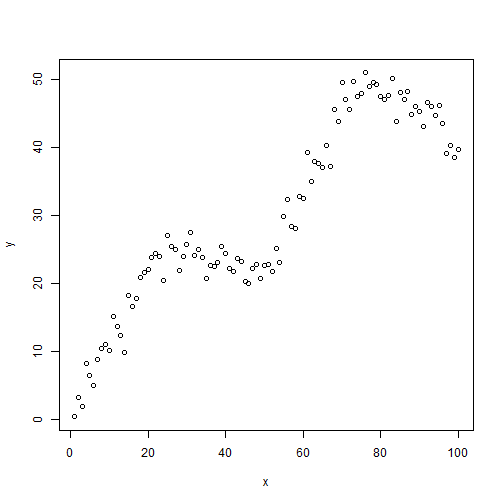It's clear there is a relationship between x and y, but the relationship is non-linear. How can we fit a linear model to this data? We could try fitting a polynomial model. The relationship seems to “change directions” four different times, so we could try fitting a 4th-degree polynomial model.

modp <- lm(y ~ poly(x, 4))
plot(x,y)
lines(x, fitted(modp))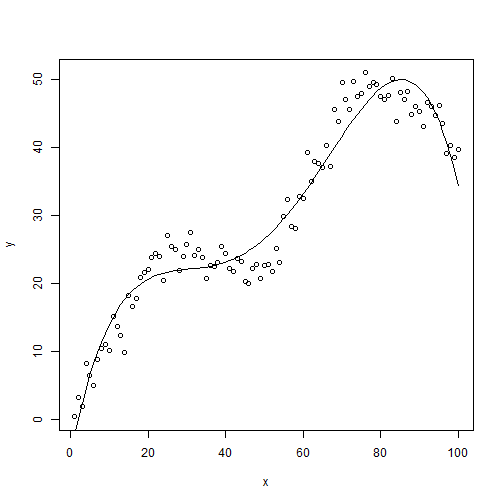This sort of captures the general nature of the relationship but the peaks and valleys just aren't quite right. They either over-predict or under-predict. We would like to do better.

Another approach might be to use a nonparametric regression approach such as loess. If we set the span parameter to 0.5, which controls the amount of smoothing, we get a decent fitting model:

modl <- loess(y ~ x, span = 0.5)
plot(x, y)
lines(x, predict(modl))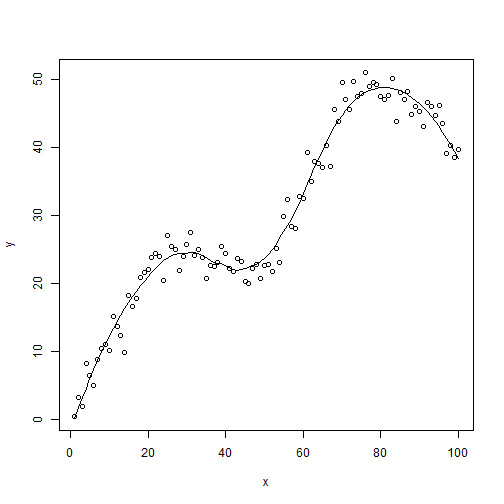This matches what we get when we use ggplot with the smooth geom:

library(ggplot2)
ggplot(data.frame(x, y), aes(x, y)) +
geom_point() +
geom_smooth(se = F, span = 0.5)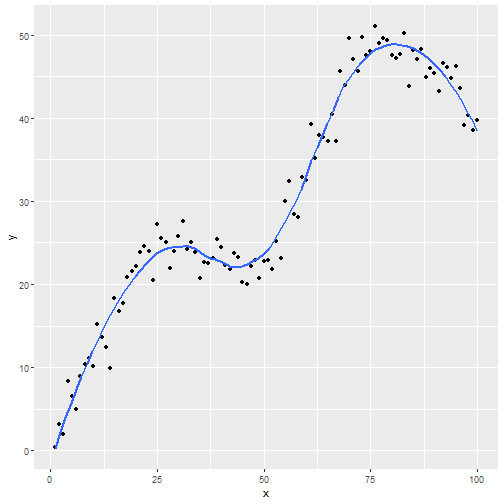But the drawback is we have no prediction equation. This is a non-parametric approach, hence no parameters were estimated.

This leads us to restricted cubic splines, or natural splines. The basic idea is to model a non-linear relationship such as the one in our example with piecewise cubic polynomials. Let's go ahead and first use natural splines in our linear model and then talk a little more about what's happening behind the scenes. Below we first load the splines package (a recommended package that comes with base R) so we have access to the ns function (natural splines). Notice we call ns on our predictor and specify df as 4. Specifying df = 4 implies 3 interior knots (ie, not including two “boundary knots”).

library(splines)
modns <- lm(y ~ ns(x, df = 4))
plot(x, y)
lines(x, predict(modns))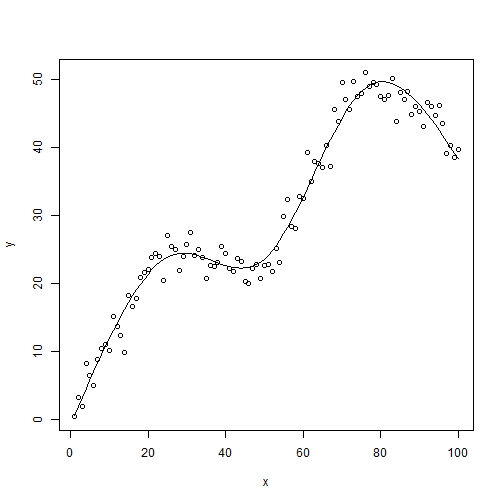This looks better than the polynomial model. And unlike the loess fit, we have a prediction equation:

summary(modns)

##
## Call:
## lm(formula = y ~ ns(x, df = 4))
##
## Residuals:
##    Min     1Q Median     3Q    Max
## -6.355 -1.302 -0.052  1.279  5.325
##
## Coefficients:
##                Estimate Std. Error t value Pr(>|t|)
## (Intercept)      0.6489     0.8242   0.787    0.433
## ns(x, df = 4)1  11.9996     1.0508  11.420   <2e-16 ***
## ns(x, df = 4)2  50.1753     1.0438  48.071   <2e-16 ***
## ns(x, df = 4)3  72.0807     2.1079  34.195   <2e-16 ***
## ns(x, df = 4)4  19.5918     0.9794  20.004   <2e-16 ***
## ---
## Signif. codes:  0 '***' 0.001 '**' 0.01 '*' 0.05 '.' 0.1 ' ' 1
##
## Residual standard error: 2.114 on 95 degrees of freedom
## Multiple R-squared:  0.977,  Adjusted R-squared:  0.976
## F-statistic:  1008 on 4 and 95 DF,  p-value: < 2.2e-16


Obviously the coefficients defy interpretation, but we can work with them as we would any other linear model. For example, we can test for linearity, that is $$H_0: \beta_2 = \beta_3 = \beta_4 = 0$$. In our model that means testing that the last 3 coefficients are equal to 0. The car package provides the powerful linearHypothesis function for this purpose.

library(car)
linearHypothesis(modns, names(coef(modns))[3:5])

## Linear hypothesis test
##
## Hypothesis:
## ns(x, df = 4)2 = 0
## ns(x, df = 4)3 = 0
## ns(x, df = 4)4 = 0
##
## Model 1: restricted model
## Model 2: y ~ ns(x, df = 4)
##
##   Res.Df     RSS Df Sum of Sq      F    Pr(>F)
## 1     98 18311.8
## 2     95   424.4  3     17887 1334.7 < 2.2e-16 ***
## ---
## Signif. codes:  0 '***' 0.001 '**' 0.01 '*' 0.05 '.' 0.1 ' ' 1


It's no surprise that the test is highly significant. We can also verify our model with natural splines is superior to the polynomial model via AIC. (Recall a lower AIC is better.)

AIC(modp, modns)

##       df      AIC
## modp   6 521.2002
## modns  6 440.3375


Now that we have played with natural splines, let's back up and try to get a better understanding of what's going on. To begin with, here's how we generated the data:

x <- 1:100         # independent variable
k <- c(25, 50, 75) # 3 interior knots

# function to construct variables x2, x3, x4
u <- function(x)ifelse(x > 0, x, 0)

x2 <- u(x - k)
x3 <- u(x - k)
x4 <- u(x - k)

# generate data
set.seed(1)
y <- 0.8 + 1*x + -1.2*x2 + 1.4*x3 + -1.6*x4 + rnorm(100,sd = 2.2)
plot(x, y)Our first predictor is x, which is simply the numbers 1 – 100. Next we define 3 “knots” at 25, 50, and 75. We then use those knots to construct three additional variables: x2, x3, and x4.

• x2 is equal to x – 25 when x – 25 is greater than 0
• x3 is equal to x – 50 when x – 50 is greater than 0
• x4 is equal to x – 75 when x – 75 is greater than 0

Finally we generate our dependent variable, y, as a function of x, x2, x3, and x4 plus some noise from a Normal(0, 2.2) distribution. The formula on the right side of the assignment operator is our True model. This is technically called a linear spline function. The best linear model we could fit to the data would be the following:

mod <- lm(y ~ x + x2 + x3 + x4)
summary(mod)

##
## Call:
## lm(formula = y ~ x + x2 + x3 + x4)
##
## Residuals:
##     Min      1Q  Median      3Q     Max
## -5.0699 -1.2797  0.1007  1.2322  4.9155
##
## Coefficients:
##             Estimate Std. Error t value Pr(>|t|)
## (Intercept)  1.34673    0.77485   1.738   0.0854 .
## x            0.97506    0.04425  22.035   <2e-16 ***
## x2          -1.14818    0.07094 -16.186   <2e-16 ***
## x3           1.35246    0.06220  21.743   <2e-16 ***
## x4          -1.57494    0.06865 -22.941   <2e-16 ***
## ---
## Signif. codes:  0 '***' 0.001 '**' 0.01 '*' 0.05 '.' 0.1 ' ' 1
##
## Residual standard error: 2.01 on 95 degrees of freedom
## Multiple R-squared:  0.9792, Adjusted R-squared:  0.9783
## F-statistic:  1117 on 4 and 95 DF,  p-value: < 2.2e-16

plot(x, y)
lines(x, fitted(mod))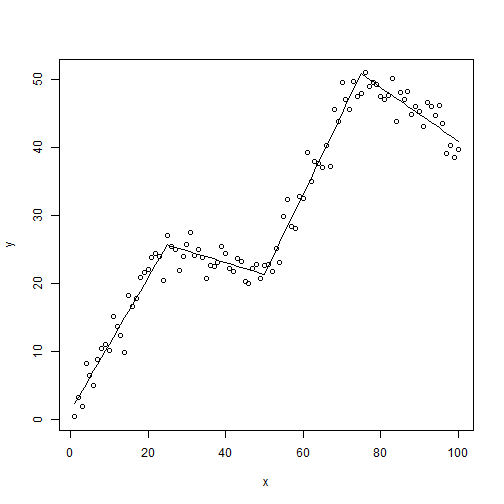So we see that to make the trajectory of our data change directions 4 times, we needed to create 4 predictors, 3 of which were based on one. This might make intuitive sense if we think of a simple parabola. It changes directions twice and has two coefficients for the $$x$$ and $$x^2$$ parameters. It's a 2nd degree polynomial. Likewise for a 3rd degree polynomial, a 4th degree polynomial, and so forth. There's only one $$x$$, but the trajectory of $$y$$ changes depending on the degree of the polynomial.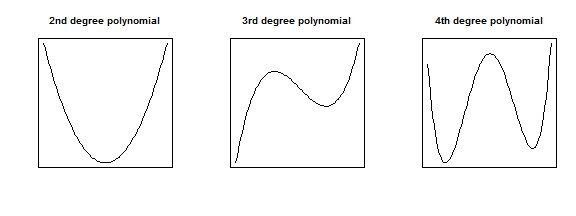The takeaway is that when we see that our response variable has a non-linear relationship with a predictor variable in real life, we may need to consider more than just a single slope coefficient to model the relationship. In the example we've been using the slope, or trajectory, changes directions 4 times, which suggests using four predictors instead of one. We tried a 4th degree polynomial of $$x$$ but saw that didn't work as well as we would have liked. A recommended approach then is to try natural splines.

The basic, and I mean very basic, idea of natural splines is to fit a 3rd degree polynomial to data within knots, and then connect those lines together. For example, below is our data with knots defined at 0, 25, 50, 75, and 100.

plot(x,y)
abline(v = c(0,25,50,75,100))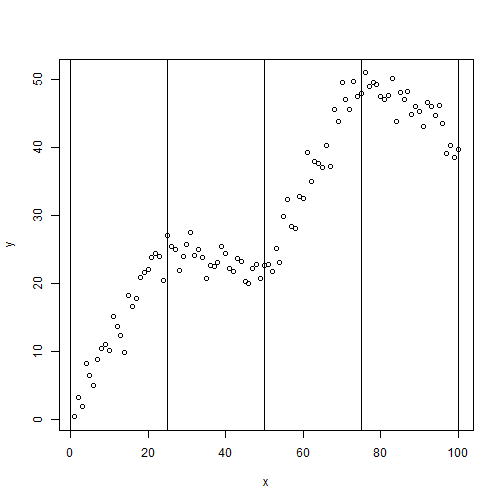With 5 knots, we have 4 regions of data. Within those 4 regions of data, natural splines essentially allow us to fit 4 different 3rd degree polynomials, all smoothed together. The magic is in how the 3 additional predictors are generated. Using the ns function in the splines package, we can create a basis matrix that allows us to fit a natural cubic spline using regular regression functions such as lm and glm. How the basis matrix is generated is quite complicated and probably something you'll just want to take on faith, like I do.

We can sort of see the natural spline in action if we fit and then color the lines between the knots. Below we regress $$y$$ on a natural spline of $$x$$ with knots defined at 25, 50 and 70. We then color the fitted lines differently between the knots.

plot(x,y)
mod.ns <- lm(y ~ ns(x, knots = c(25,50,75)))
lines(x[1:25], fitted(mod.ns)[1:25],col=1)
lines(x[26:50], fitted(mod.ns)[26:50],col=2)
lines(x[51:75], fitted(mod.ns)[51:75],col=3)
lines(x[76:100], fitted(mod.ns)[76:100],col=4)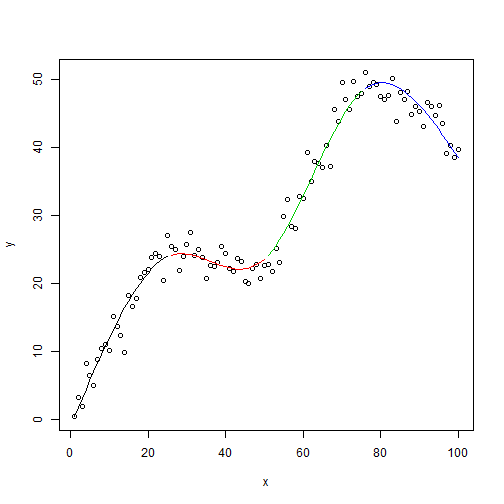Notice the red and green interior lines have a noticeable 3rd degree polynomial shape. The exterior red and blue lines look more like a 2nd degree polynomial. That's because natural splines are constrained to be linear in the tails (ie, the boundary knots). For this reason, natural splines are sometimes called restricted cubic splines. If we didn't want that constraint, we could use the bs function to generate a b-spline matrix basis. Here's what that looks like.

plot(x,y)
mod.bs <- lm(y ~ bs(x, knots = c(25,50,75)))
lines(x[1:25], fitted(mod.bs)[1:25],col=1)
lines(x[26:50], fitted(mod.bs)[26:50],col=2)
lines(x[51:75], fitted(mod.bs)[51:75],col=3)
lines(x[76:100], fitted(mod.bs)[76:100],col=4)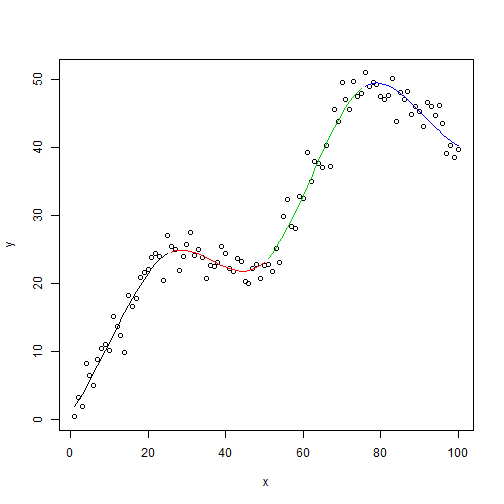Notice the blue line in particular curls up at the boundary as would be expected of a 3rd degree polynomial. But that's not the only difference. Look at the summary output. Notice the bs function generated matrix with 6 columns instead of 4.

summary(mod.bs)

##
## Call:
## lm(formula = y ~ bs(x, knots = c(25, 50, 75)))
##
## Residuals:
##     Min      1Q  Median      3Q     Max
## -5.8247 -1.1582 -0.0468  1.2780  5.0283
##
## Coefficients:
##                               Estimate Std. Error t value Pr(>|t|)
## (Intercept)                      1.983      1.218   1.628  0.10699
## bs(x, knots = c(25, 50, 75))1    6.623      2.281   2.903  0.00461 **
## bs(x, knots = c(25, 50, 75))2   33.328      1.529  21.794  < 2e-16 ***
## bs(x, knots = c(25, 50, 75))3    8.281      1.840   4.501 1.96e-05 ***
## bs(x, knots = c(25, 50, 75))4   60.284      1.722  35.000  < 2e-16 ***
## bs(x, knots = c(25, 50, 75))5   40.697      1.913  21.278  < 2e-16 ***
## bs(x, knots = c(25, 50, 75))6   38.377      1.688  22.736  < 2e-16 ***
## ---
## Signif. codes:  0 '***' 0.001 '**' 0.01 '*' 0.05 '.' 0.1 ' ' 1
##
## Residual standard error: 2.052 on 93 degrees of freedom
## Multiple R-squared:  0.9788, Adjusted R-squared:  0.9774
## F-statistic: 714.2 on 6 and 93 DF,  p-value: < 2.2e-16


So fitting a b-spline means actually fitting a more complicated model. For this reason and the fact that b-splines can be poory behaved in the tails (Harrell, p. 24), most statisticians recommend working with natural splines.

To wrap up, let's go over some guidelines for using natural splines with real data. First off, you don't want to go looking at your data and guessing how many times it changes direction to determine your knots or degrees of freedom! I did that simply as a way to help explain how splines worked. In practice you'll want to determine degrees of freedom based on sample size and how important you suspect a predictor to be. Harrell states that 4 degrees of freedom is usually sufficient. If your sample is on the small side, perhaps choose 3 degrees of freedom. If it's large, go with 5. And notice we're talking about degrees of freedom, not knots. The location of knots isn't that crucial and ns will automatically select knots based on the quantiles of the predictor.

Something else to remember is that the coefficients on a model with natural splines defy any sort of interpretation. So forget using the “1-unit increase in x leads to a __ increase in y” method to explain association. An alternative approach is an effect plot, which allows you to visualize your model given certain predictor values. Here's a quick demonstration using a simplified example that comes with the powerful effects package. Below we model the log of prestige, a prestige score for someone's occupation, as a function of logged income and a 4 degree of freedom natural spline basis of education. Calling summary and anova on the model object will reveal the natural spline appears warranted and highly significant.

library(effects)
mod.pres1 <- lm(log(prestige) ~ log(income) + ns(education, 4),
data=Prestige)


But what does it mean? What is the association between prestige and education when, say, holding income at the mean value? An effect plot sheds some light.

eff.log <- Effect("education", mod.pres1, transformation=list(inverse=exp))
plot(eff.log)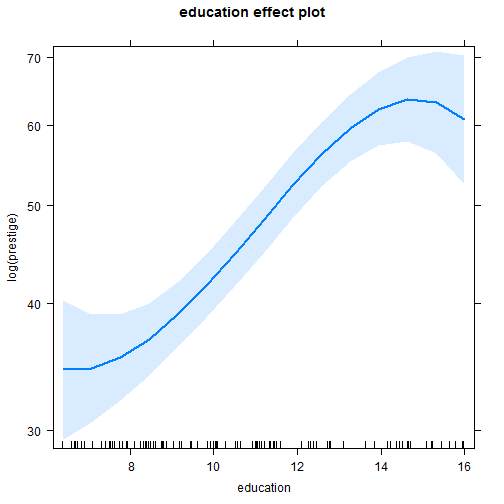This shows that from about 9 – 14, the effect of education is pretty dramatic on prestige scores, but rather uncertain in the extremes, below 9 and above 14. From 10 – 14, it looks like a 2-level increase in education is worth about a 10 point increase in prestige scores. We couldn't guess that from the summary output but we can sort of infer it from the effect plot. Again, this is just an example and not a replication of the original analysis.

Reference:

F. Harrell. Regression Modeling Strategies: With Applications to Linear Models, Logistic and Ordinal Regression, and Survival Analysis. 2nd Ed Springer. 2015

# Recreating a Geometric CDF plot from Casella and Berger

For reasons I no longer remember I decided a while back to reproduce Figure 1.5.2 from Casella and Berger (page 32):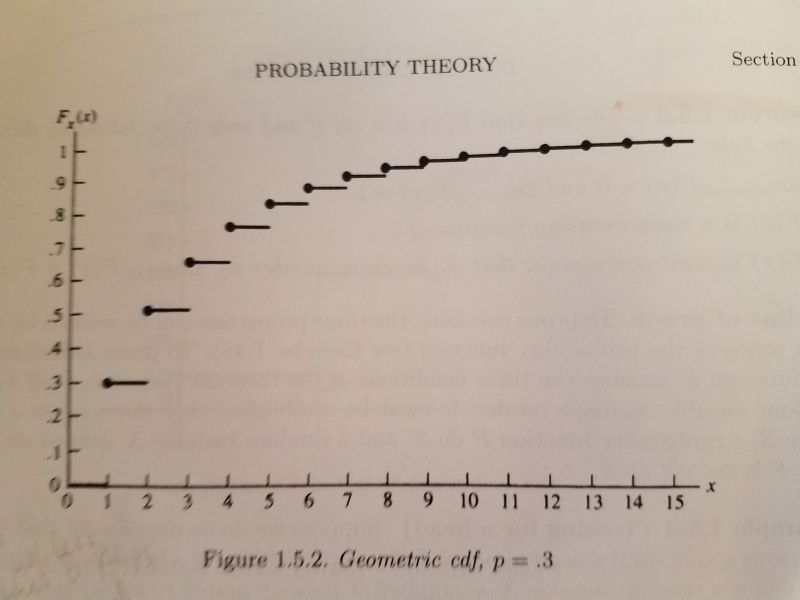It's a plot of the cumulative distribution function of a geometric distribution with p = 0.3. The geometric distribution is best explained with coin-flipping. Let's say we keep flipping a coin until we observe a head. Now let X be a random variable that equals the number of tosses required to see a head. So if we get a head on the first flip of the coin, that means we needed one toss. If it takes two tosses, we got a tail on the first toss and then a head on the second toss. The graph above visualizes the possibilities for 15 flips with probability of getting heads set to 0.3. We see that the probability of taking only one toss is 0.3, which makes sense since the probability of getting heads is 0.3. The probability of requiring two tosses is about 0.5. And so on. The straight lines indicate the probabilities only change at the whole numbers. There is no such thing as, say, 2.23 flips. This is often referred to as a step function. We see that there's a high probability of getting a head by the 5th or 6th flip. This is a simple waiting-time distribution that can be used to model the number of successes before a failure and vice-versa.

To recreate this plot in R I used the pgeom function, which returns the cumulative probability of the geometric distribution for a given number of failures before a success occurs and a given probability of success. For example, the probability of 0 failures before observing a success with p = 0.3:

pgeom(0, prob = 0.3)

##  0.3


The probability of 1 failure before observing a success with p = 0.3:

pgeom(1, prob = 0.3)

##  0.51


To generate all the probabilities in the plot, we can do the following:

y <- pgeom(q = 0:14, prob = 0.3)


Notice that instead of using 1:15 (number of total flips for success), we use 0:14. That's because pgeom works with the number of failures before success. Now that we have our y coordinates, we can create our first version of the plot as follows:

plot(x = 1:15, y, pch = 19)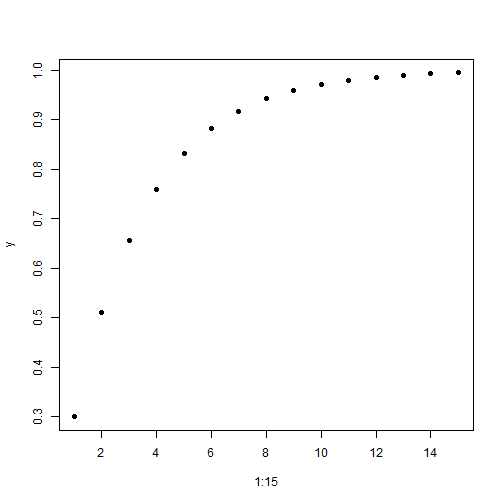Now let's add the horizontal lines using the segments function:

plot(x = 1:15, y, pch = 19)
segments(x0 = 0:15, y0 = c(0, y),
x1 = 1:16, y1 = c(0, y), lwd = 2)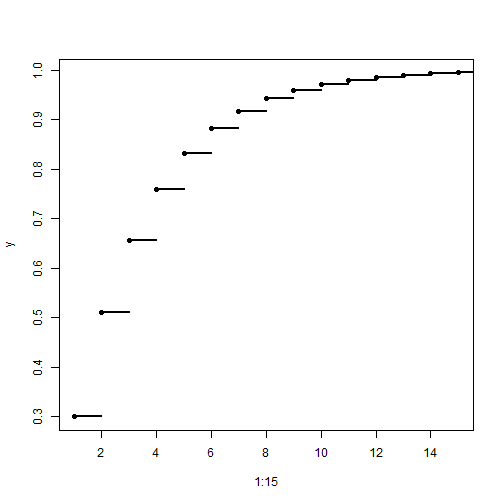The x0 and y0 coordinates are where the line starts. The x1 and y1 coordinates are where the line ends. Since the lines are horizontal, the y coordinates are the same for the start and end postions. The y coordinates include 0, so we add that value to y with the c function. The lwd = 2 argument makes the line a little thicker and darker.

Our plot is basically done, but just for fun I wanted to see how close I could make it look like the version in the book. That means relabeling the axes, moving the axis labels to the ends, and removing the lines at the top and right side of the plot. It also means moving the axis tick marks inside the plotting area. After some trial and error and deep dives into R documentation, here's what I was able to come up with:

plot(x = 1:15, y, pch = 19,
yaxt = "n", xaxt = "n", ylim = c(0,1.05), xlim = c(0,15.5),
bty="l", xaxs="i", yaxs = "i", xlab = "", ylab = "")
segments(x0 = 0:15, y0 = c(0, y),
x1 = 1:16, y1 = c(0, y), lwd = 2)
axis(side = 1, at = 0:15,
labels = 0:15, tcl = 0.5, family = "serif")
axis(side = 2, at = seq(0,1,0.1),
labels = c(0,paste(".",1:9),1), las=1, tcl = 0.5, family = "serif")
mtext(text = expression(italic(x)), side = 4,
at = 0, las = 1, line = 0.5, family = "serif")
mtext(text = expression(italic(F[x](x))), side = 3,
at = 0, line = 0.5, family = "serif")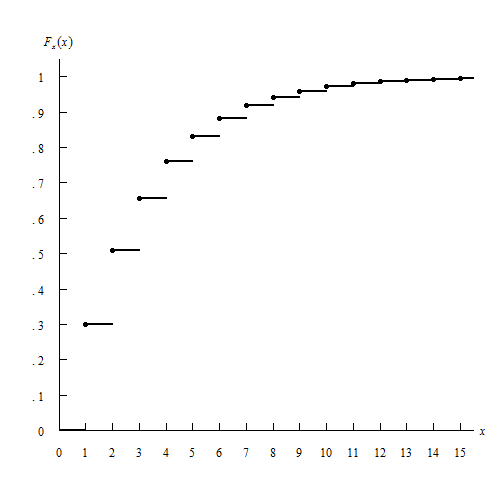In the plot function:

• the yaxt = "n" and xaxt = "n" arguments say “don't label the axes”. I instead use the axis function to create the axes.
• the ylim = c(0,1.05) and xlim = c(0,15.5) arguments tell the axes to end at 1.05 and 15.5, respectively. I wanted them to extend beyond the last value just as they do in the book.
• the bty="l" argument says “draw a box around the plot like a capital letter L”
• the xaxs="i" and yaxs = "i" arguments ensures the axes fit within the original range of the data. The default is to extend the range by 4 percent at each end. Again, I was trying to recreate the graph in the book. Notice how the origin has the x-axis and y-axis 0 values right next to one another.
• The xlab = "" and ylab = "" set blank axis labels. I instead use the mtext function to add axis labels.

The segments function remained unchanged.

The axis function allows us to explicitly define how the axis is created.

• The side argument specifies which side of the plot we're placing the axis. 1 is the bottom, 2 is the left.
• at is where we draw the tick marks.
• labels are how we label the tick marks.
• The tcl argument specifies how long to make the tick marks and in what direction. A positive value extends the tick marks into the plotting region.
• The las argument in the second axis function makes the labels on the y-axis horizontal.

Finally I used the mtext function to create the axis labels. mtext writes text into the margins of a plot and can take some trial and error to get the placement of text just so.

• The text argument is what text we want to place in the graph. In this case I make use of the expression function which allows us to create mathematical notation. For example, the syntax expression(italic(F[x](x))) returns $$F_x (x)$$
• The side argument again refers to where in the plot to place the text. 3 is top and 4 is right. This means the y-axis label is actually in the top of the plot and the x-axis label is on the right. A little bit of a hack.
• at says where to place the text along the axis parallel to the margin. In both cases we use 0. We want the y-axis label at the 0 point corresponding to the x-axis, and the x-axis label at the 0 point corresponding to the y-axis. A little confusing, I think.
• The las argument rotates the x label to be horizontal
• The line argument specifies on which margin line to place the text, starting at 0 counting outwards. This is one that takes some trial and error to get just right.
• The family argument specifies the type of font to use. “serif” is like Times New Roman.

Not perfect, but close enough. Of course I much prefer the R defaults when it comes to plotting layout. Even though R allows us to recreate this graph, I don't think it's necessarily a “good” graph.

I also decided to tackle this using ggplot. Here's how far I got.

library(ggplot2)
dat <- data.frame(x = 1:15, y = pgeom(q = 0:14, prob = 0.3))
dat2 <- data.frame(x = 0:15, y = c(0, dat$y[-16]), xend = 1:16, yend = c(0,dat$y[-16]))

ggplot(dat, aes(x = x, y = y)) + geom_point() +
geom_segment(aes(x = x, y = y, xend = xend, yend = yend), data = dat2) +
scale_x_continuous(breaks = 0:15, labels = 0:15) +
scale_y_continuous(breaks = seq(0,1,0.1), labels = c(0,paste(".",1:9),1)) +
labs(x = "x", y = expression(italic(F[x](x)))) +
theme(panel.background = element_blank(),
axis.line.x = element_line(color = "black"),
axis.line.y = element_line(color = "black"),
axis.title.x = element_text(face = "italic", hjust = 1),
axis.title.y = element_text(face = "italic", hjust = 1, angle = 0))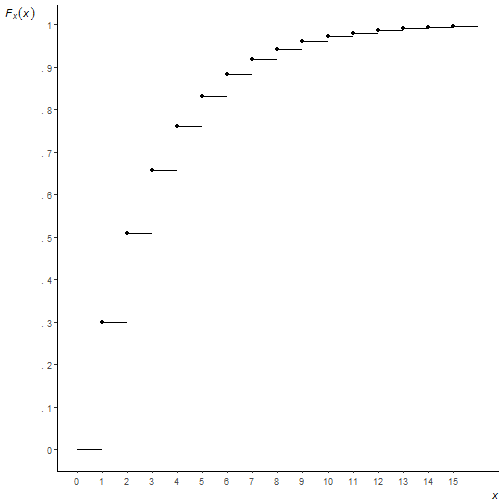You can see I couldn't figure out how to move the axis ticks into the plotting region, or how to place axis labels at the ends of the axis, or how to get the origin to start at precisely (0, 0). I'm not saying it can't be done, just that I lost interest in trying to go further. And a very strong argument can be made that these are things you shouldn't do anyway! But as I said at the outset, this was all for fun.

# Revisiting some old R-code

One of my first blog posts on this site simulated rolling a die 6 times and observing if side i was observed on the ith roll at least once. (For example, rolling a 3 on my 3rd roll would be a success.) I got the idea from one of my statistics textbooks which had a nice picture of the simulation converging to the true probability of 0.665 over the course of 500 trials. I was able to recreate the simulation in R and I guess I got excited and blogged about it. I was reminded of this today when I opened that old textbook and came across the R code that I had actually written in the margin by hand. Apparently I was super proud of my ground-breaking R code and decided to preserve it for posterity in writing. smh

Over 5 years later my precious R code looks pretty juvenile. It’s way more complicated than it needs to be and doesn’t take advantage of R's vectorized calculations. As Venables and Ripley say in MASS, “Users coming to S from other languages are often slow to take advantage of the power of S to do vectorized calculations…This often leads to unnecessary loops.” Indeed. I'm living proof of that.

I shouldn’t be too hard on my myself though. I was not yet familiar with functions like replicate and cumsum. And I was more focused on recreating the plot than writing optimal R code. I went with what I knew. And R, so forgiving and flexible, accommodated my novice enthusiasm.

Here is how I would approach the problem today:

r.out <- replicate(n = 500, any(sample(1:6, size = 6, replace = T) == 1:6))
p.out <- cumsum(r.out)/seq(500)
plot(x = seq(500), y = p.out, type = "l", ylim = c(0,1),
main = "Convergence to probability as n increases", xlab = "n")
abline(h = 0.665)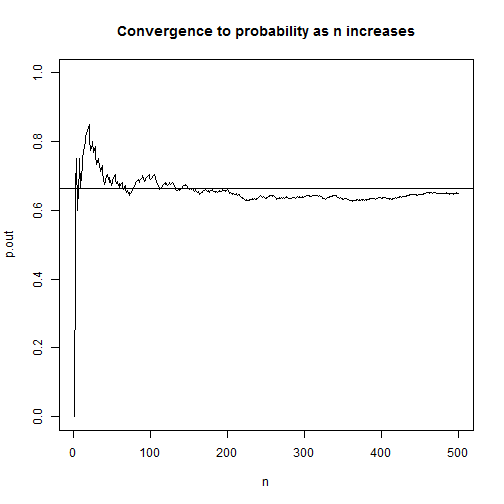On line 1, we use the sample function to “roll a die 6 times” by sampling the numbers 1 – 6, with replacement, 6 times. Then we compare the 6 results with the vector of numbers 1 – 6 using the == operator and use the any function to check if any are TRUE. Next we replicate that 500 times and store the result in r.out. This is a vector of TRUE/FALSE values which R treats numerically as 1 and 0. This means we can use cumsum to find the cumulative sum of successes. To determine the cumulative proportion of successes, we divide each cumulative sum by the trial number. The result is a vector of proportions that should start converging to 0.665. Finally we plot using base R plot and abline.

This is more efficient than my original attempt 5 years ago and better captures the spirit of the simulation. I'm sure 5 years from now if I stumble upon this post I'll have yet another more elegant way to do it. I'm already looking at it thinking, “I should have generalized this with a function, and used ggplot2 to make the graph. And I shouldn't do seq(500) twice.” In fact I know I could have avoided the replicate function by using the fact that there's a probability of $$\frac{1}{6}$$ of observing side i on the ith roll of a die. So I could have used a single rbinom call to do the simulation, like so:

r.out2 <- cumsum(rbinom(n = 500, size = 6, prob = 1/6) > 0)
p.out2 <- r.out2/seq(500)
plot(x = seq(500), y = p.out2, type = "l", ylim = c(0,1),
main = "Convergence to probability as n increases", xlab = "n")
abline(h = 0.665)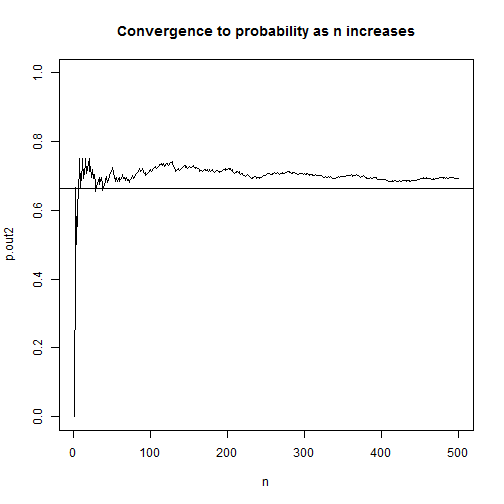In this version instead of simulating 6 literal die rolls, we simulate the number of successes in 6 die rolls. We turn each roll of the die into a binomial event: success or failure. The rbinom function allows us to simulate binomial events where size is the number of trials (or rolls in this case) and prob is the probability of success at each trial. So rbinom(n = 1, size = 6, prob = 1/6) would return a number ranging 0 to 6 indicating the number of success. Think of it as flipping 6 coins, each with probability of getting heads as $$\frac{1}{6}$$, and then counting the number of heads we observed. Setting the n argument to 500 replicates it 500 times. After that it's simply a matter of logically checking which outcomes were greater than 0 and using cumsum on the resulting TRUE/FALSE vector.

This version is way faster. I mean way faster. Compare the time it takes it to do each 1,000,000 times:

system.time({
r.out <- replicate(n = 1e6, any(sample(1:6, size = 6, replace = T) == 1:6))
p.out <- cumsum(r.out)/seq(1e6)
})

##    user  system elapsed
##    5.26    0.00    5.26

system.time({
r.out2 <- cumsum(rbinom(n = 1e6, size = 6, prob = (1/6)) > 0)
p.out2 <- r.out2/seq(1e6)
})

##    user  system elapsed
##    0.06    0.00    0.06


It's not even close. Who was the dummy that wrote that first version with replicate?

But does the new faster version reflect the experimental setting better? Not really. Remember, we're demonstrating probability concepts with die rolls in the first chapter of an intro stats textbook. That's probably not the best time to break out rbinom. And the demo was for 500 trials, not 1,000,000. I had to ramp up the trials to see the speed difference. Maybe the “right” R code in this situation is not the fastest version but rather the one that's easier to understand.

# Profile likelihood ratio confidence intervals

When you fit a generalized linear model (GLM) in R and call confint on the model object, you get confidence intervals for the model coefficients. But you also get an interesting message:

Waiting for profiling to be done...

What's that all about? What exactly is being profiled? Put simply, it's telling you that it's calculating a profile likelihood ratio confidence interval.

The typical way to calculate a 95% confidence interval is to multiply the standard error of an estimate by some normal quantile such as 1.96 and add/subtract that product to/from the estimate to get an interval. In the context of GLMs, we sometimes call that a Wald confidence interval.

Another way to determine an upper and lower bound of plausible values for a model coefficient is to find the minimum and maximum value of the set of all coefficients that satisfy the following:

$-2\log\left(\frac{L(\beta_{0}, \beta_{1}|y_{1},…,y_{n})}{L(\hat{\beta_{0}}, \hat{\beta_{1}}|y_{1},…,y_{n})}\right) < \chi_{1,1-\alpha}^{2}$

Inside the parentheses is a ratio of likelihoods. In the denominator is the likelihood of the model we fit. In the numerator is the likelihood of the same model but with different coefficients. (More on that in a moment.) We take the log of the ratio and multiply by -2. This gives us a likelihood ratio test (LRT) statistic. This statistic is typically used to test whether a coefficient is equal to some value, such as 0, with the null likelihood in the numerator (model without coefficient, that is, equal to 0) and the alternative or estimated likelihood in the denominator (model with coefficient). If the LRT statistic is less than $$\chi_{1,0.95}^{2} \approx 3.84$$, we fail to reject the null. The coefficient is statisically not much different from 0. That means the likelihood ratio is close to 1. The likelihood of the model without the coefficient is almost as high the model with it. On the other hand, if the ratio is small, that means the likelihood of the model without the coefficient is much smaller than the likelihood of the model with the coefficient. This leads to a larger LRT statistic since it's being log transformed, which leads to a value larger than 3.84 and thus rejection of the null.

Now in the formula above, we are seeking all such coefficients in the numerator that would make it a true statement. You might say we're “profiling” many different null values and their respective LRT test statistics. Do they fit the profile of a plausible coefficient value in our model? The smallest value we can get without violating the condition becomes our lower bound, and likewise with the largest value. When we're done we'll have a range of plausible values for our model coefficient that gives us some indication of the uncertainly of our estimate.

Let's load some data and fit a binomial GLM to illustrate these concepts. The following R code comes from the help page for confint.glm. This is an example from the classic Modern Applied Statistics with S. ldose is a dosing level and sex is self-explanatory. SF is number of successes and failures, where success is number of dead worms. We're interested in learning about the effects of dosing level and sex on number of worms killed. Presumably this worm is a pest of some sort.

# example from Venables and Ripley (2002, pp. 190-2.)
ldose <- rep(0:5, 2)
numdead <- c(1, 4, 9, 13, 18, 20, 0, 2, 6, 10, 12, 16)
sex <- factor(rep(c("M", "F"), c(6, 6)))
budworm.lg <- glm(SF ~ sex + ldose, family = binomial)
summary(budworm.lg)

##
## Call:
## glm(formula = SF ~ sex + ldose, family = binomial)
##
## Deviance Residuals:
##      Min        1Q    Median        3Q       Max
## -1.10540  -0.65343  -0.02225   0.48471   1.42944
##
## Coefficients:
##             Estimate Std. Error z value Pr(>|z|)
## (Intercept)  -3.4732     0.4685  -7.413 1.23e-13 ***
## sexM          1.1007     0.3558   3.093  0.00198 **
## ldose         1.0642     0.1311   8.119 4.70e-16 ***
## ---
## Signif. codes:  0 '***' 0.001 '**' 0.01 '*' 0.05 '.' 0.1 ' ' 1
##
## (Dispersion parameter for binomial family taken to be 1)
##
##     Null deviance: 124.8756  on 11  degrees of freedom
## Residual deviance:   6.7571  on  9  degrees of freedom
## AIC: 42.867
##
## Number of Fisher Scoring iterations: 4


The coefficient for ldose looks significant. Let's determine a confidence interval for the coefficient using the confint function. We call confint on our model object, budworm.lg and use the parm argument to specify that we only want to do it for ldose:

confint(budworm.lg, parm = "ldose")

## Waiting for profiling to be done...

##     2.5 %    97.5 %
## 0.8228708 1.3390581


We get our “waiting” message though there really was no wait. If we fit a larger model and request multiple confidence intervals, then there might actually be a waiting period of a few seconds. The lower bound is about 0.8 and the upper bound about 1.32. We might say every increase in dosing level increase the log odds of killing worms by at least 0.8. We could also exponentiate to get a CI for an odds ratio estimate:

exp(confint(budworm.lg, parm = "ldose"))

## Waiting for profiling to be done...

##    2.5 %   97.5 %
## 2.277027 3.815448


The odds of “success” (killing worms) is at least 2.3 times higher at one dosing level versus the next lower dosing level.

To better understand the profile likelihood ratio confidence interval, let's do it “manually”. Recall the denominator in the formula above was the likelihood of our fitted model. We can extract that with the logLik function:

den <- logLik(budworm.lg)
den

## 'log Lik.' -18.43373 (df=3)


The numerator was the likelihood of a model with a different coefficient. Here's the likelihood of a model with a coefficient of 1.05:

num <- logLik(glm(SF ~ sex + offset(1.05*ldose), family = binomial))
num

## 'log Lik.' -18.43965 (df=2)


Notice we used the offset function. That allows us to fix the coefficient to 1.05 and not have it estimated.

Since we already extracted the log likelihoods, we need to subtract them. Remember this rule from algebra?

$\log\frac{M}{N} = \log M – \log N$

So we subtract the denominator from the numerator, multiply by -2, and check if it's less than 3.84, which we calculate with qchisq(p = 0.95, df = 1)

-2*(num - den)

## 'log Lik.' 0.01184421 (df=2)

-2*(num - den) < qchisq(p = 0.95, df = 1)

##  TRUE


It is. 1.05 seems like a plausible value for the ldose coefficient. That makes sense since the estimated value was 1.0642. Let's try it with a larger value, like 1.5:

num <- logLik(glm(SF ~ sex + offset(1.5*ldose), family = binomial))
-2*(num - den) < qchisq(p = 0.95, df = 1)

##  FALSE


FALSE. 1.5 seems too big to be a plausible value for the ldose coefficient.

Now that we have the general idea, we can program a while loop to check different values until we exceed our threshold of 3.84.

cf <- budworm.lg$coefficients # fitted coefficient 1.0642 cut <- qchisq(p = 0.95, df = 1) # about 3.84 e <- 0.001 # increment to add to coefficient LR <- 0 # to kick start our while loop while(LR < cut){ cf <- cf + e num <- logLik(glm(SF ~ sex + offset(cf*ldose), family = binomial)) LR <- -2*(num - den) } (upper <- cf)  ## ldose ## 1.339214  To begin we save the original coefficient to cf, store the cutoff value to cut, define our increment of 0.001 as e, and set LR to an initial value of 0. In the loop we increment our coefficient estimate which is used in the offset function in the estimation step. There we extract the log likelihood and then calculate LR. If LR is less than cut (3.84), the loop starts again with a new coefficient that is 0.001 higher. We see that our upper bound of 1.339214 is very close to what we got above using confint (1.3390581). If we set e to smaller values we'll get closer. We can find the LR profile lower bound in a similar way. Instead of adding the increment we subtract it: cf <- budworm.lg$coefficients  # reset cf
LR <- 0                           # reset LR
while(LR < cut){
cf <- cf - e
num <- logLik(glm(SF ~ sex + offset(cf*ldose), family = binomial))
LR <- -2*(num - den)
}
(lower <- cf)

##    ldose
## 0.822214


The result, 0.822214, is very close to the lower bound we got from confint (0.8228708).

This is a very basic implementation of calculating a likelihood ratio confidence interval. It is only meant to give a general sense of what's happening when you see that message Waiting for profiling to be done.... I hope you found it helpful. To see how R does it, enter getAnywhere(profile.glm) in the console and inspect the code. It's not for the faint of heart.

I have to mention the book Analysis of Categorical Data with R, from which I gained a better understanding of the material in this post. The authors have kindly shared their R code at the following web site if you want to have a look: http://www.chrisbilder.com/categorical/

To see how they “manually” calculate likelihood ratio confidence intervals, go to the following R script and see the section “Examples of how to find profile likelihood ratio intervals without confint()”: http://www.chrisbilder.com/categorical/Chapter2/Placekick.R

# Earthquake data and Benford’s Law

Much has been written about Benford's Law, that weird phenonmenon where if you have a naturally occuring set of numerical data, 30% of the numbers will begin with 1, 18% will begin with 2, 12% will begin with 3, and so on. You might expect the distribution of leading digits to be uniformly distributed, but no, that just isn't the case. 60% of the time the leading digit is 1, 2, or 3. Though it may seem like some curious mathematical anomaly, Benford's Law has been used to detect fraud in elections and accounting.

In this post let's use R to verify that earthquake data obtained from the USGS does indeed follow Benford's Law. On May 28, 2016, I downloaded data for all earthquakes in the past 30 days. The data has a number of fields, including earthquake magnitude, depth of the earthquake, location, time and many others. Benford's Law says those numbers should follow a particular distribution.

The formula for Benford's Law is as follows:

$P(d) = \log_{10} \left(1+\frac{1}{d}\right)$

That says the probability that a digit d occurs as the first number is equal the log base 10 of 1 + 1/d. We can quickly generate these for d = 1 – 9 in R:

log10(1 + (1/(1:9)))

##  0.30103000 0.17609126 0.12493874 0.09691001 0.07918125 0.06694679
##  0.05799195 0.05115252 0.04575749


And we can make a quick plot as follows:

barplot(log10(1 + (1/(1:9))), names.arg = 1:9, main = "Benford's Law")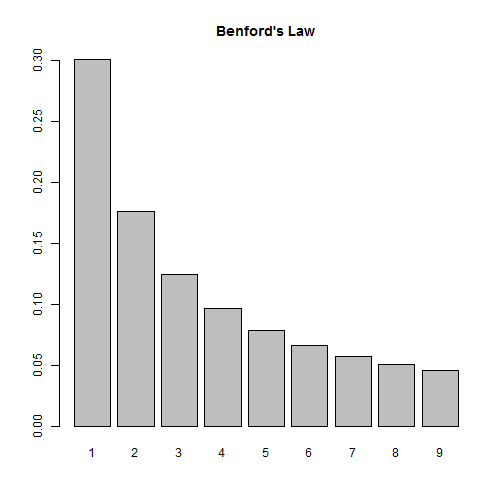So according to this law, if we look at the distribution of first digits in our earthquake data, we should see them closely follow this distribution. Let's find out!

First we need to import the data. Thanks to the USGS, this data comes ready for analysis. All we need to do is read it into R:

dat <- read.csv("all_month.csv")
nrow(dat)

##  8584


Over 8500 earthquakes in the past 30 days! A quick look at the magnitude shows us most of them are very small:

summary(dat$mag)  ## Min. 1st Qu. Median Mean 3rd Qu. Max. NA's ## -1.900 0.790 1.280 1.526 1.930 7.200 20  This also tells us we have some negative numbers (?) as well as some missing data, NA's. We also have numbers that start with 0 and have a decimal, such as 0.79. (In this case, Benford would say the number “starts” with a 7, not a 0.) This means when we determine the leading digits, we'll need to ignore negative signs, leading 0s, decimals and missing values. Let's investigate at the mag column. First we remove the NA's. Below is.na(dat$mag) generates a logical vector of TRUE and FALSE values. Adding an ! in front reverses the TRUE and FALSE values. Finally inserting the result in subsetting brackets returns only those values that are TRUE, ie not missing.

digits <- dat$mag[!is.na(dat$mag)]


Next we extract the first digits. To help with this I use two R packages, magrittr and stringr. The magrittr package allows us to “chain” commands together with the %>% operator. The stringr package provides the str_extract function that allows us to extract phrases that follow a certain pattern. So what we have below can be translated as follows:

• take the absoluet value of digits (to get rid of negative signs)
• convert digits to character (so we can use the next two functions)
• extract anything that is not a “0” or a “.” (We express this a regular expression: "[^0\\.]")
• extract the first digit (starting and ending at position 1)
library(magrittr)
library(stringr)
digits <- abs(digits) %>%
as.character() %>%
str_extract(pattern = "[^0\\.]") %>%
substr(1,1)


As an extra precaution, we then convert digits to a factor and set levels to be all digits 1 through 9. This ensure all digits are represented in subsequent calculations.

digits <- factor(digits, levels = 1:9) # ensure all digits represented


And finally we tally the first digits and calculate proportions:

table(digits) %>% prop.table()

## digits
##          1          2          3          4          5          6
## 0.42800141 0.17134139 0.05738763 0.09447248 0.05809177 0.03990142
##          7          8          9
## 0.04459570 0.05304542 0.05316277


We see 1 appearing a lot more often, but it's hard to tell how the other digits compare to Benford's Law. Let's put both distributions on the same graph. To do this, I went ahead and created a function that will allow us to check any vector of numbers against Benford's Law. Let's load the function and see how it works, then we'll break it down and explain it.

library(ggplot2)
compareBenford <- function(d){
digits <- d[!is.na(d)]
digits <- substr(stringr::str_extract(as.character(abs(digits)), pattern = "[^0\\.]"),1,1)
digits <- factor(digits, levels = 1:9) # ensure all digits represented
depth <- prop.table(table(digits))
ben <- log10(1 + (1/(1:9)))
dat2 <- data.frame(ben, depth)
names(dat2) <- c("Benford","Digit",deparse(substitute(d)))
dat2L <- reshape2::melt(dat2,id.vars="Digit", variable.name = "Type", value.name = "Frequency")
ggplot(dat2L, aes(x=Digit, y=Frequency, fill=Type)) +
geom_bar(stat = "identity", position = "dodge")
}

compareBenford(dat$mag)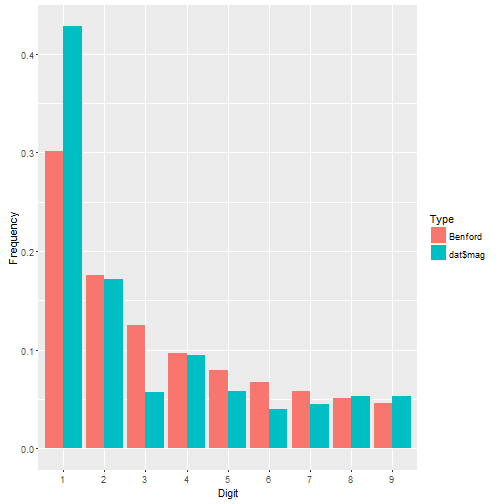We see dat$mag has more 1's than we might expect and fewer 3's, but otherwise seems to follow the distribution pretty closely. Let's check out earthquake depth.

compareBenford(dat$depth)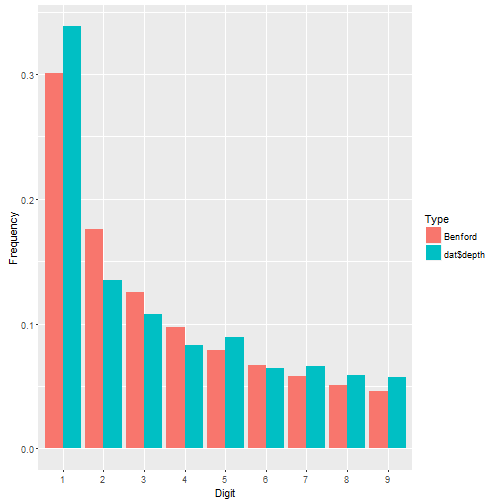This appears to fit even better. About the function: • the first four lines are what we did before. Notice I went ahead and nested the functions instead of using magrittr. That's how I originally coded it before deciding to write a blog post. Then I decided to break out magrittr for the fun of it. • After that I calculate Benford's proportions. • Then I put both sets of proportions in a data frame. • Then I change the names of the data frame. Notice there are three names to change, not two. Why? The depth vector is actually a table. When it gets pulled into a data frame, two columns are produced: the table cells and the table names. The names are the digits 1 – 9. Notice I also use deparse(substitute(d)) to name the first-digit proportions in the data frame. This ensures that whatever vector I give my function, the name of it will be the vector itself. So if I give it dat$mag, the column name of the first-digit proportions will be dat\$mag.
• Next I reshape the data using the melt function in the reshape2 package. This puts all the proportions in one column called Frequency and the type of proportion in another column called Type. I still have my Digit column that indicates which proportion goes with which digit. Having my data in this form allows me to use ggplot to map fill color to Type.
• Finally I create the graph. I set Digit on the x-axis, Frequency on the y-axis, and map Type to the fill color. The geom_bar function says draw a bar plot. The stat = "identity" and position = "dodge" arguments say set the height of the bars to the actual values and “dodge” them so they're next to one another.

Let's look at ALL numeric columns in the earthquakes data. We need to identify all numeric columns and pull them into a vector for our function to work. Here's how I do it:

theCols <- sapply(dat, class) %in% c("numeric","integer")
numCols <- names(dat)[theCols]
allDigits <- unlist(dat[,numCols])

compareBenford(allDigits)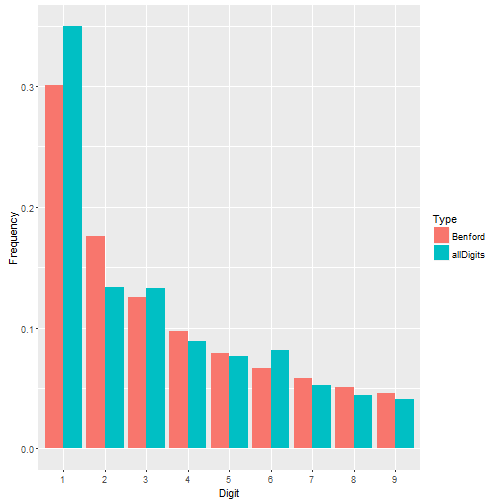Not a bad fit. Benford's Law seems to work just fine for the earthquake data.

But what about data where Benford's Law doesn't work? Recall that Benford's Law applies to most naturally occuring sets of numbers. Not all. Take the iris data that come with R. These are measurements of 50 flowers from each of 3 species of iris. This is a small data set looking at very specific measurements. It doesn't seem to follow Benford's Law very well.

irisDigits <- unlist(iris[,1:4])
compareBenford(irisDigits)